•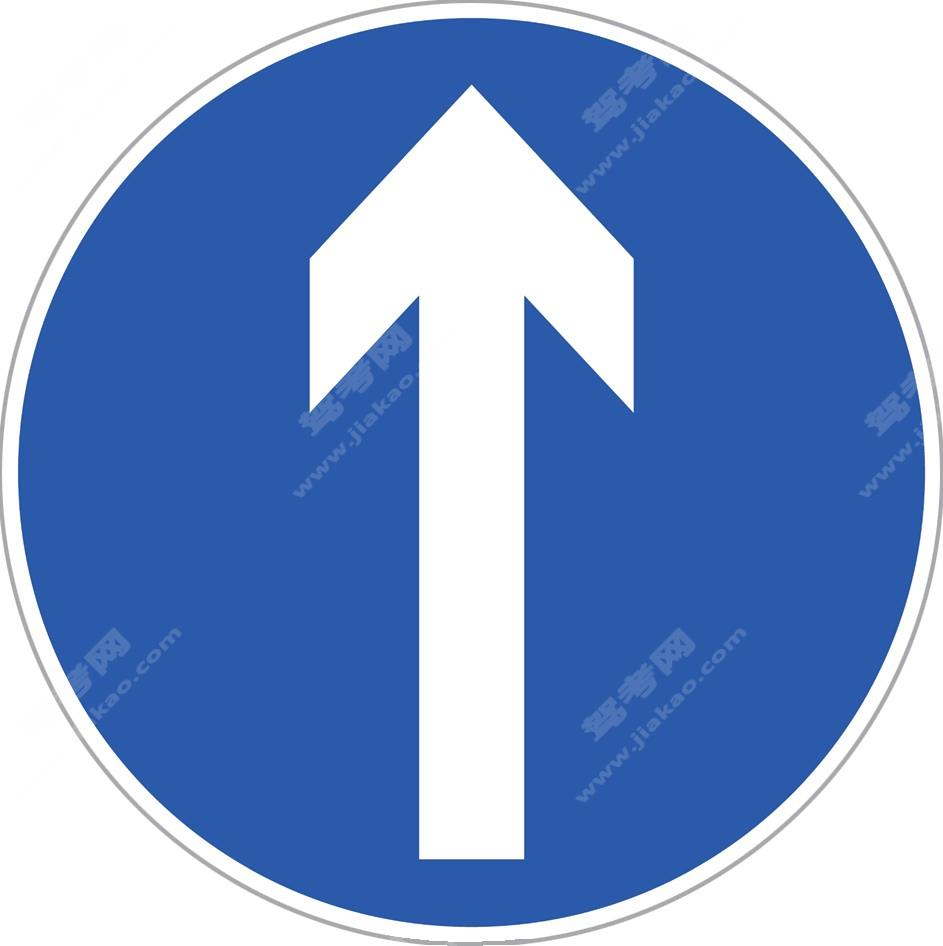直行

•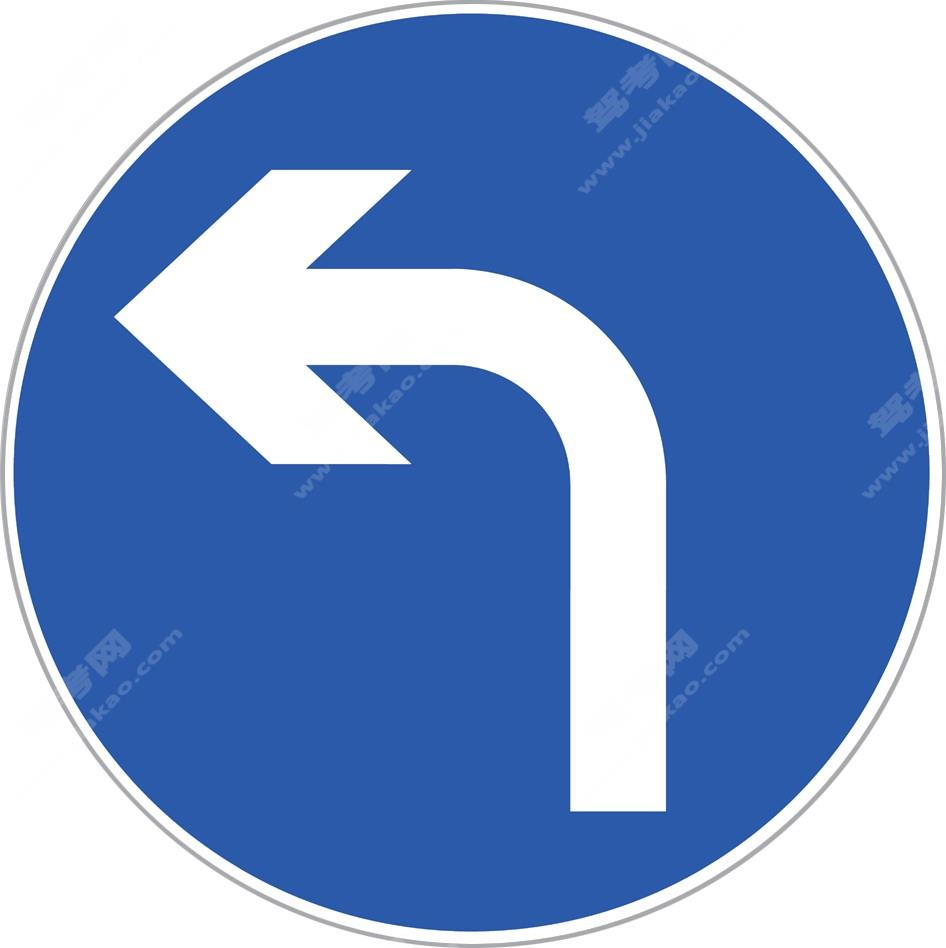向左转弯

•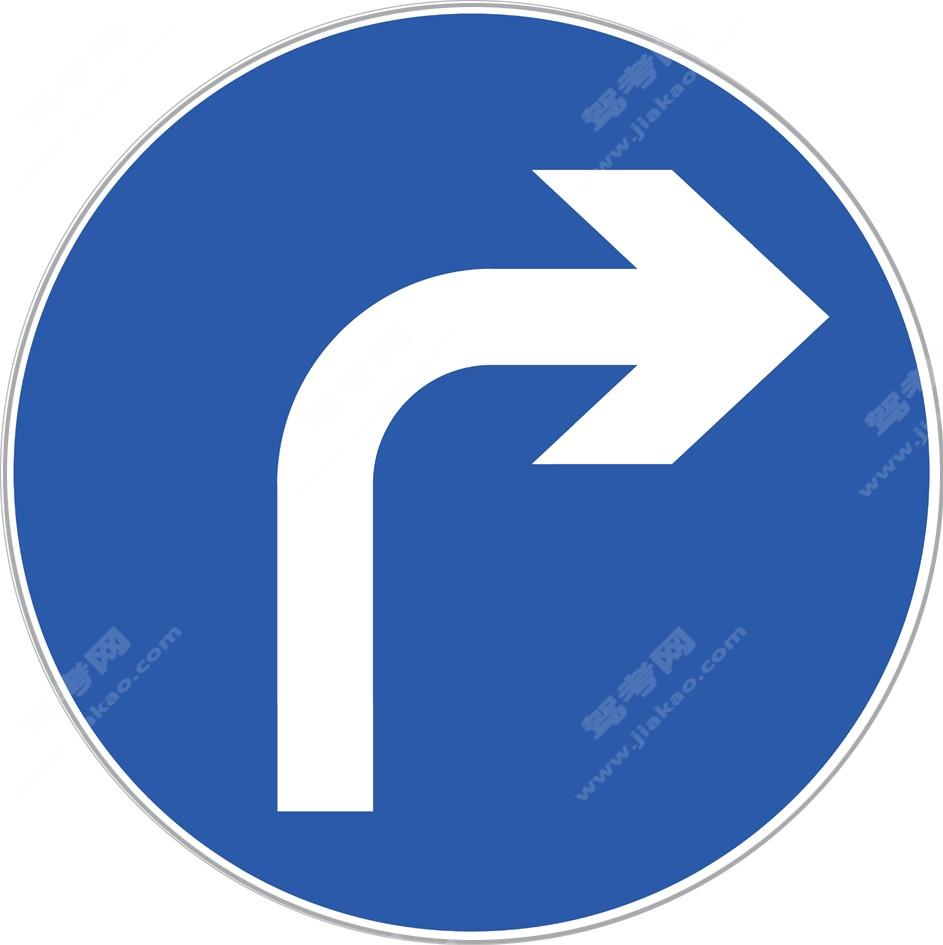向右转弯

•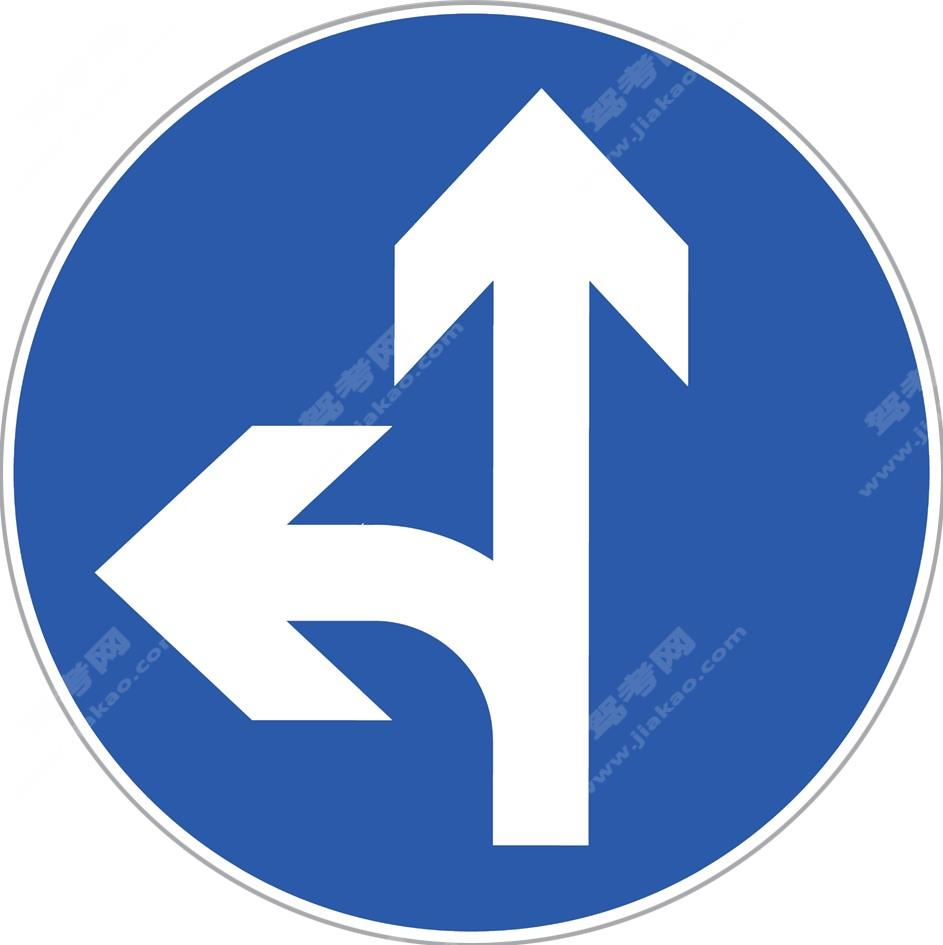直行和向左转弯

•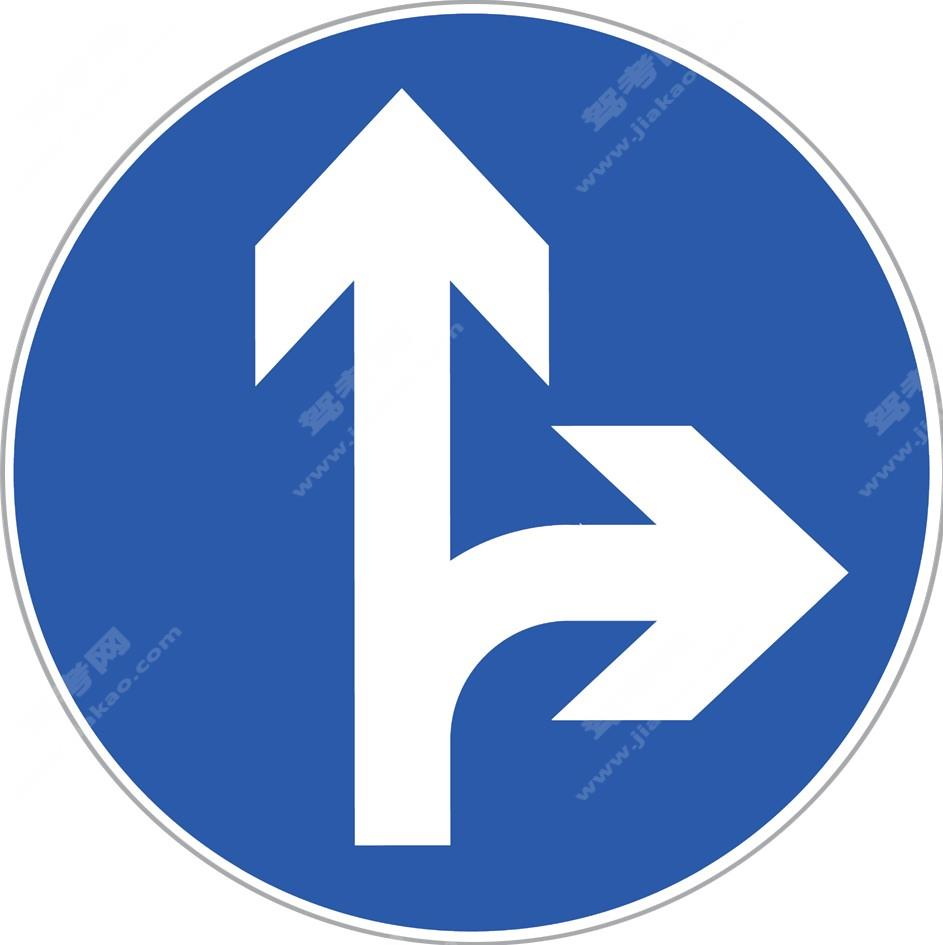直行和向右转弯

•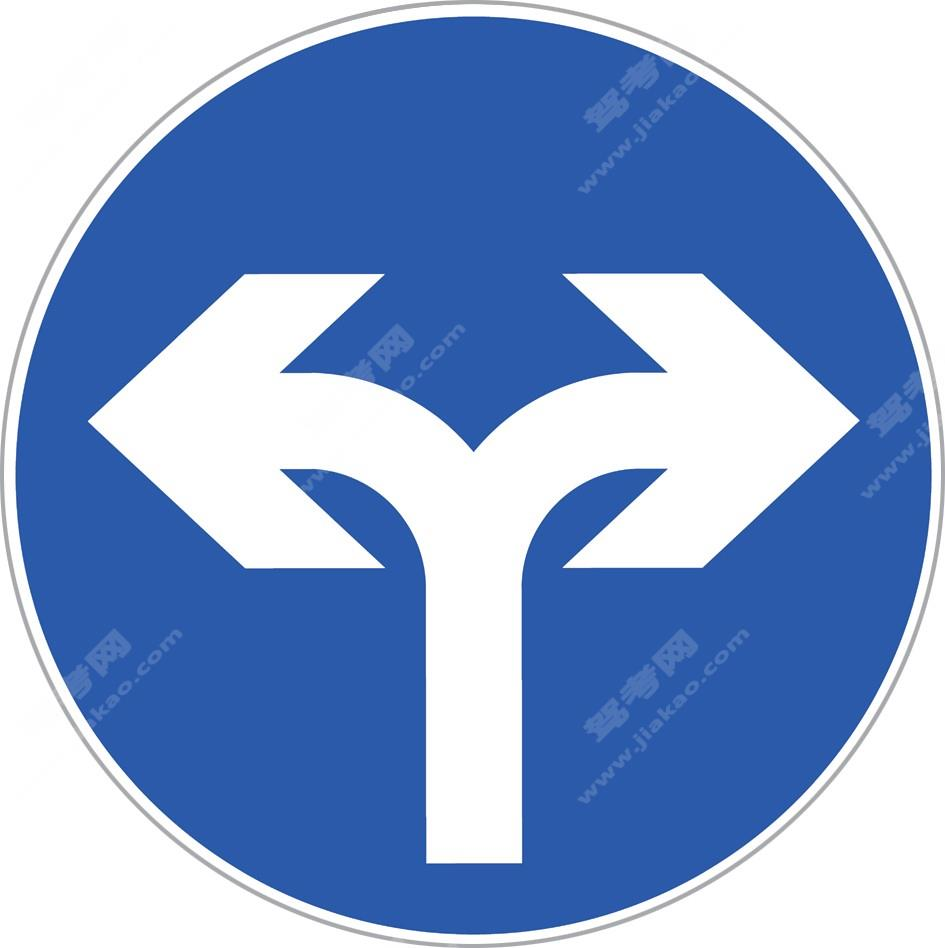向左和向右转弯

•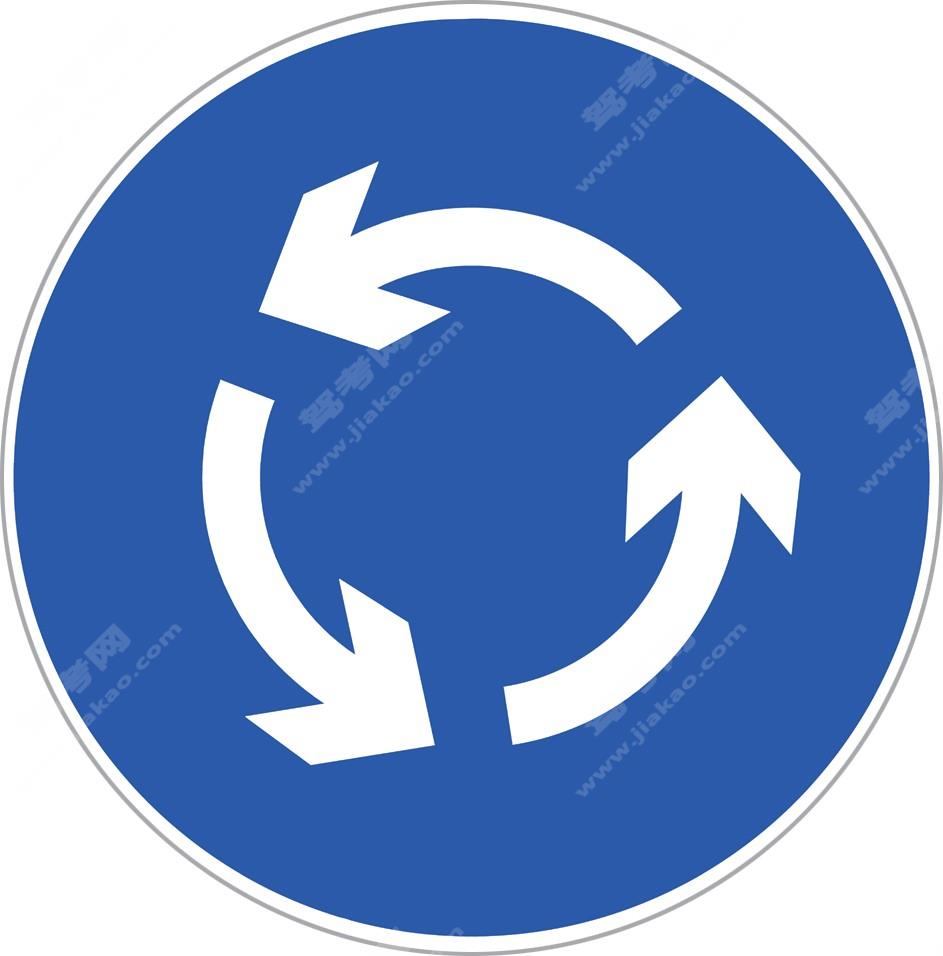环岛行驶

•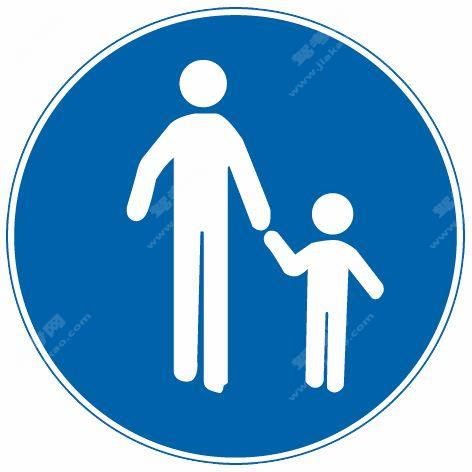步行（旧）

•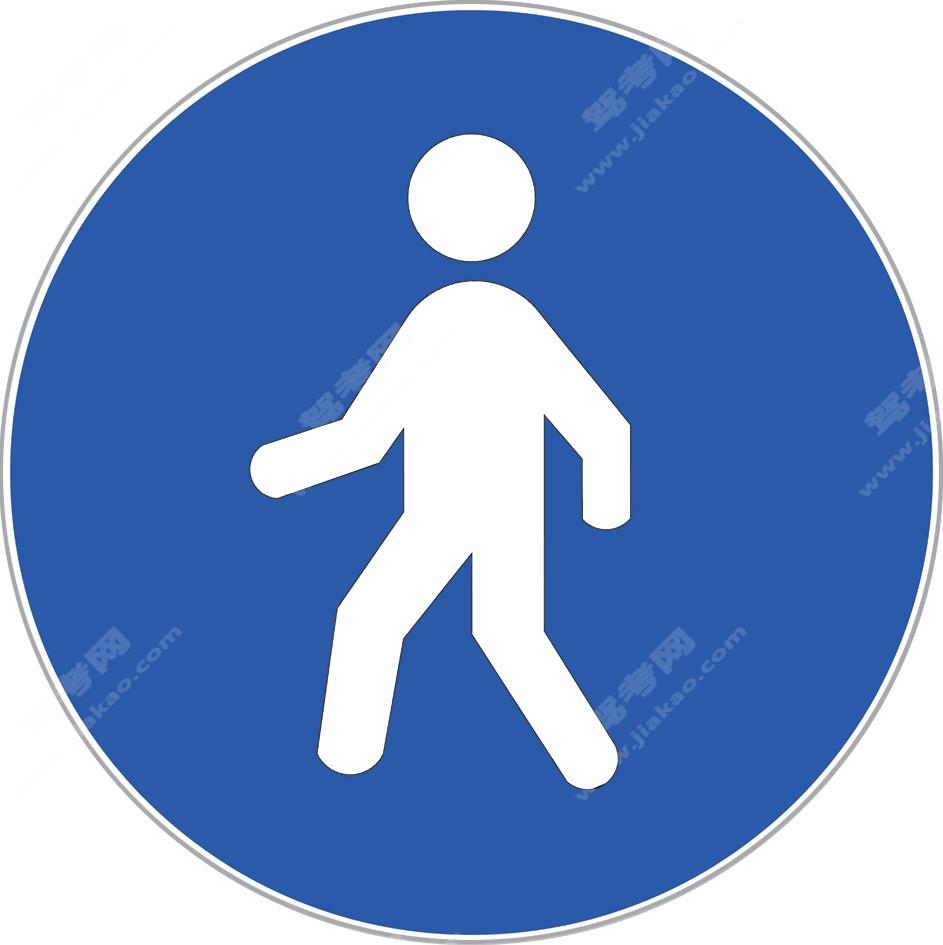行人（新）

•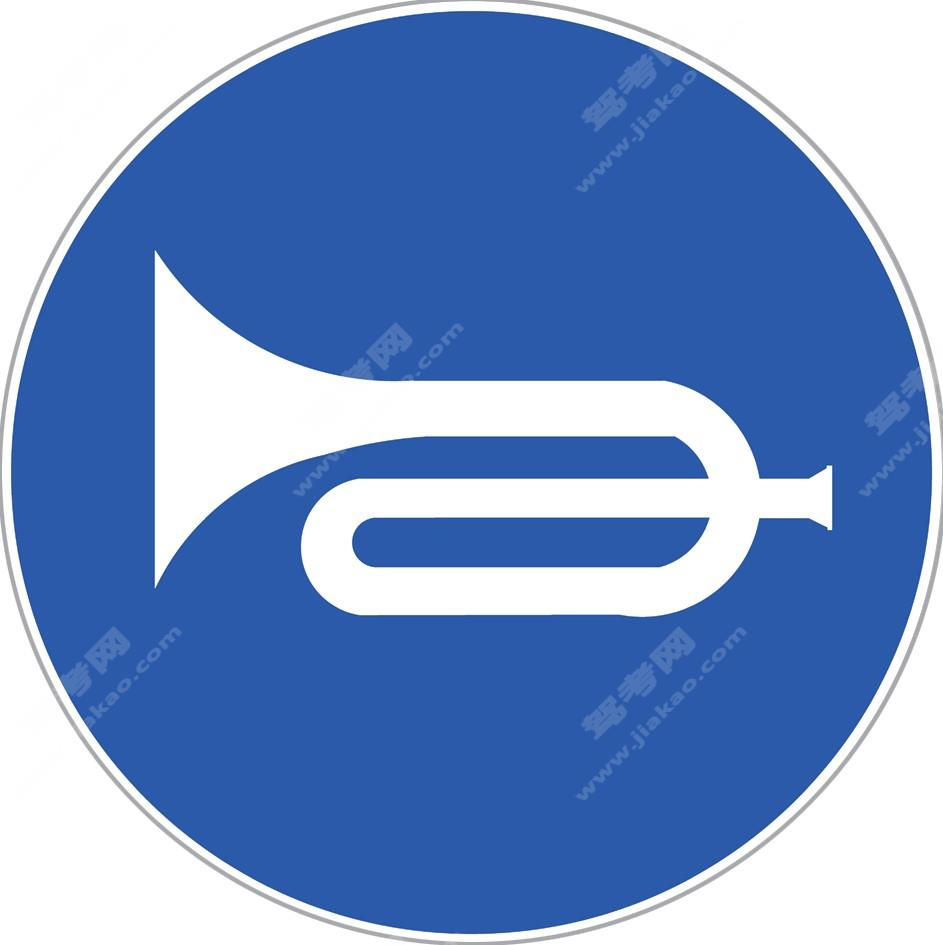鸣喇叭

•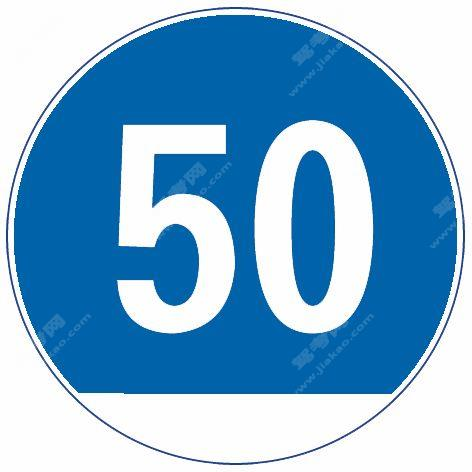最低限速（旧）

•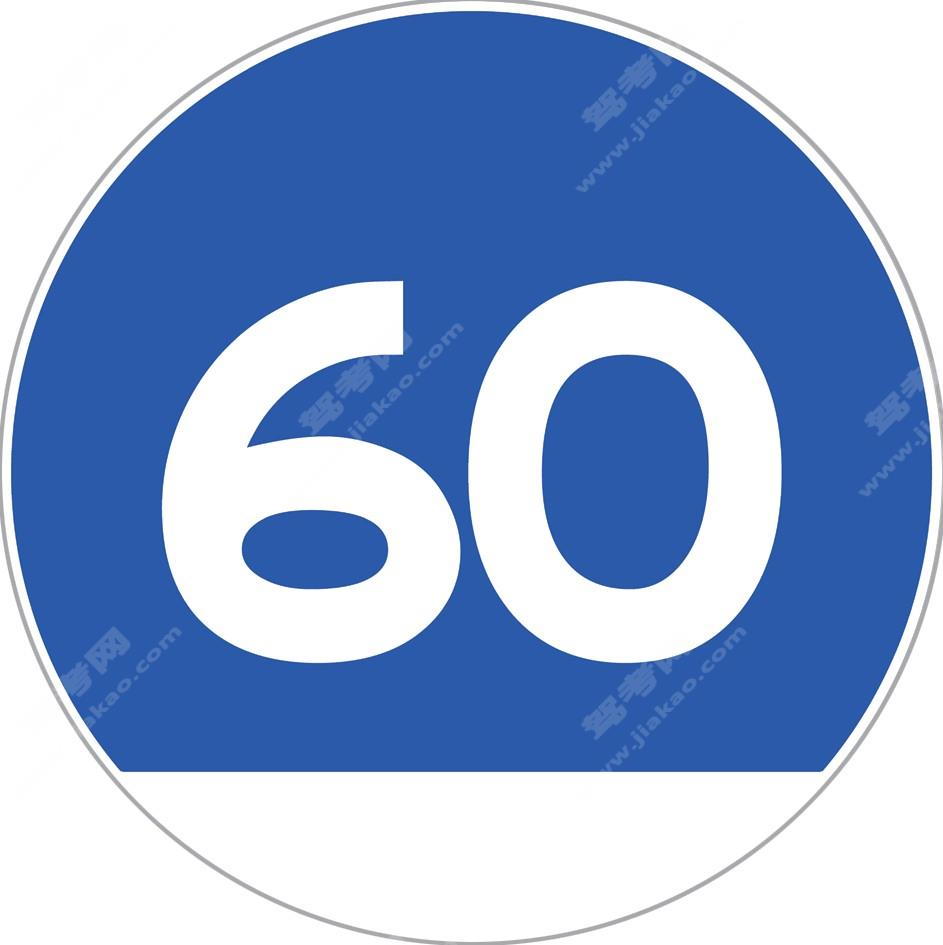最低限速（新）

•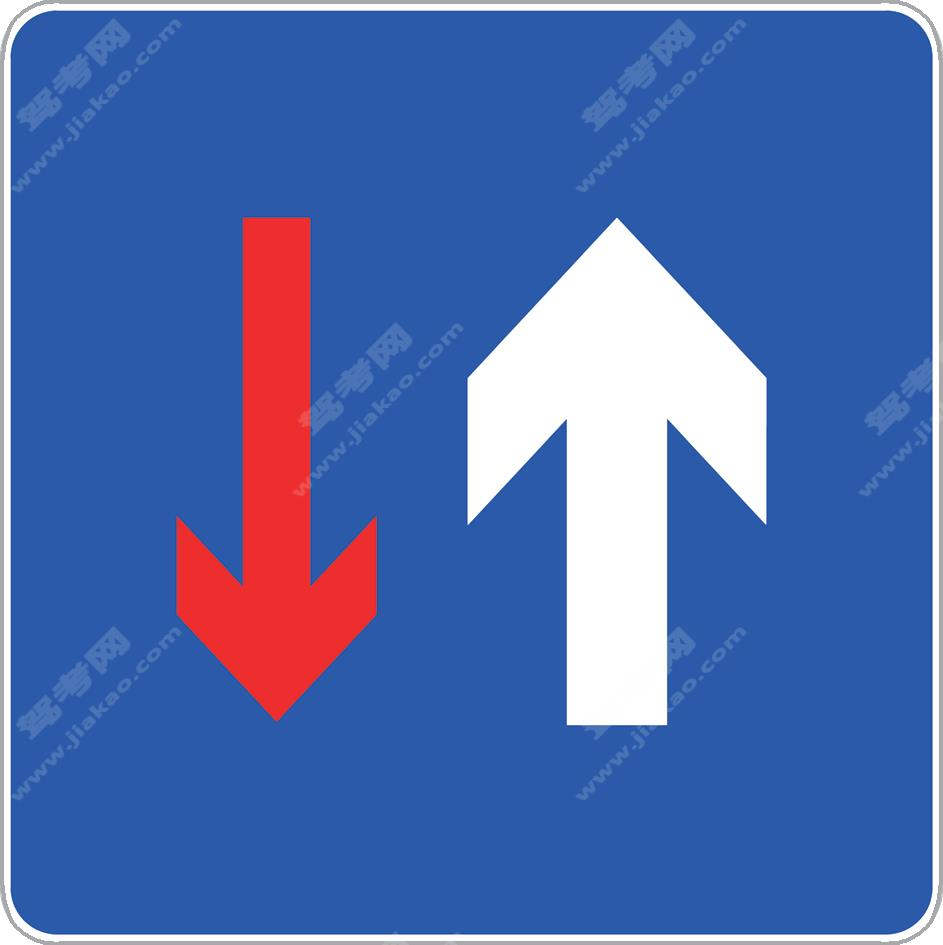会车先行

•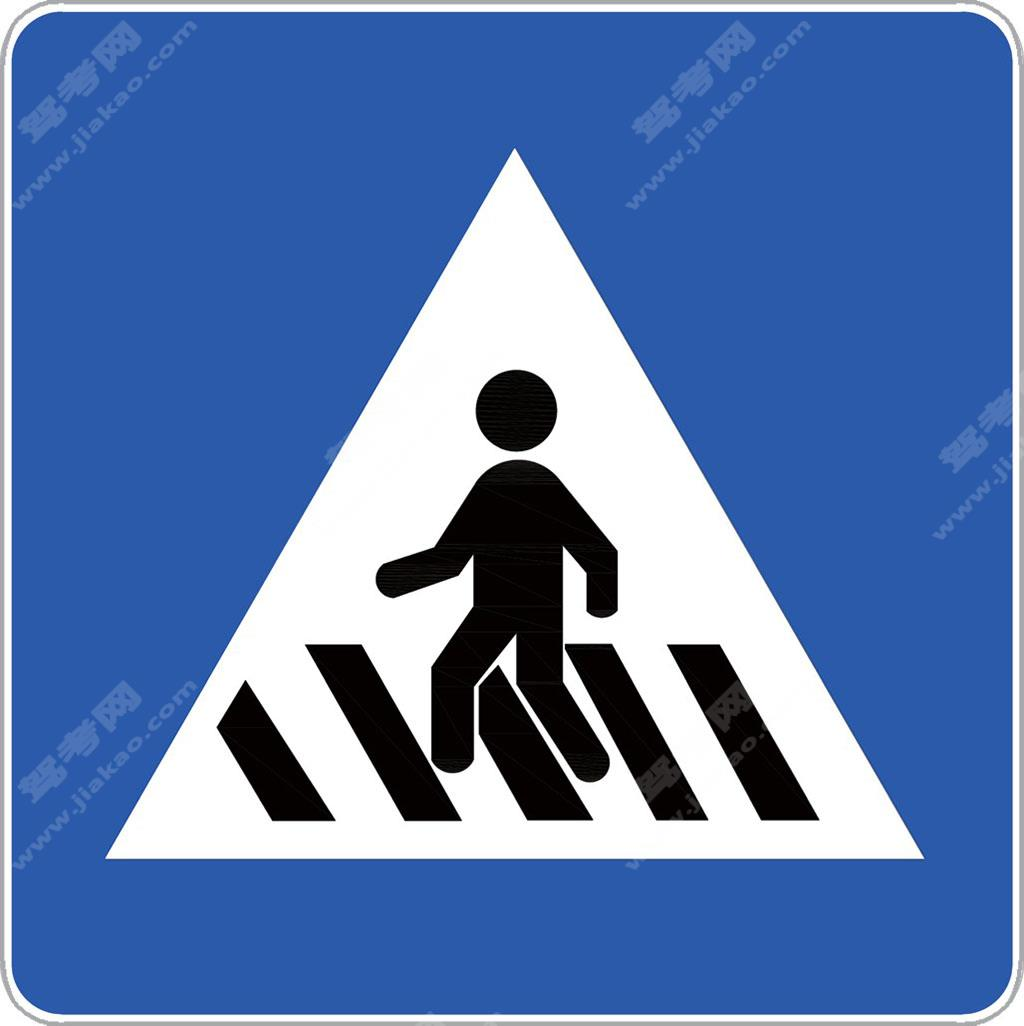人行横道

•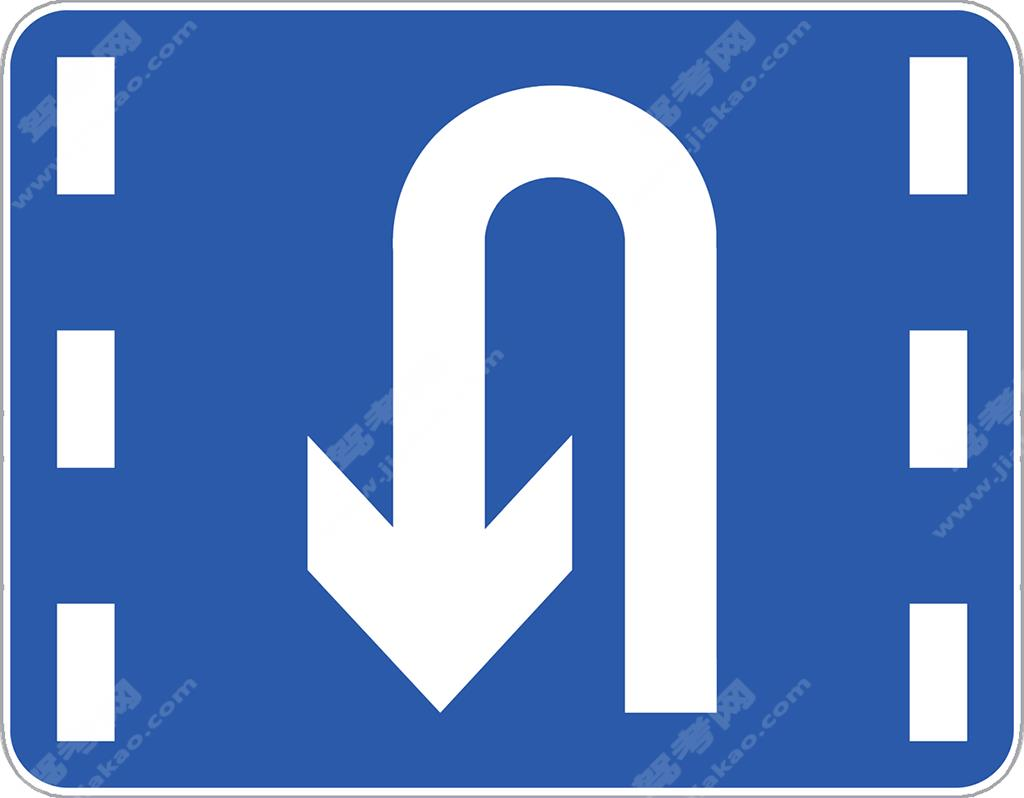掉头车道

•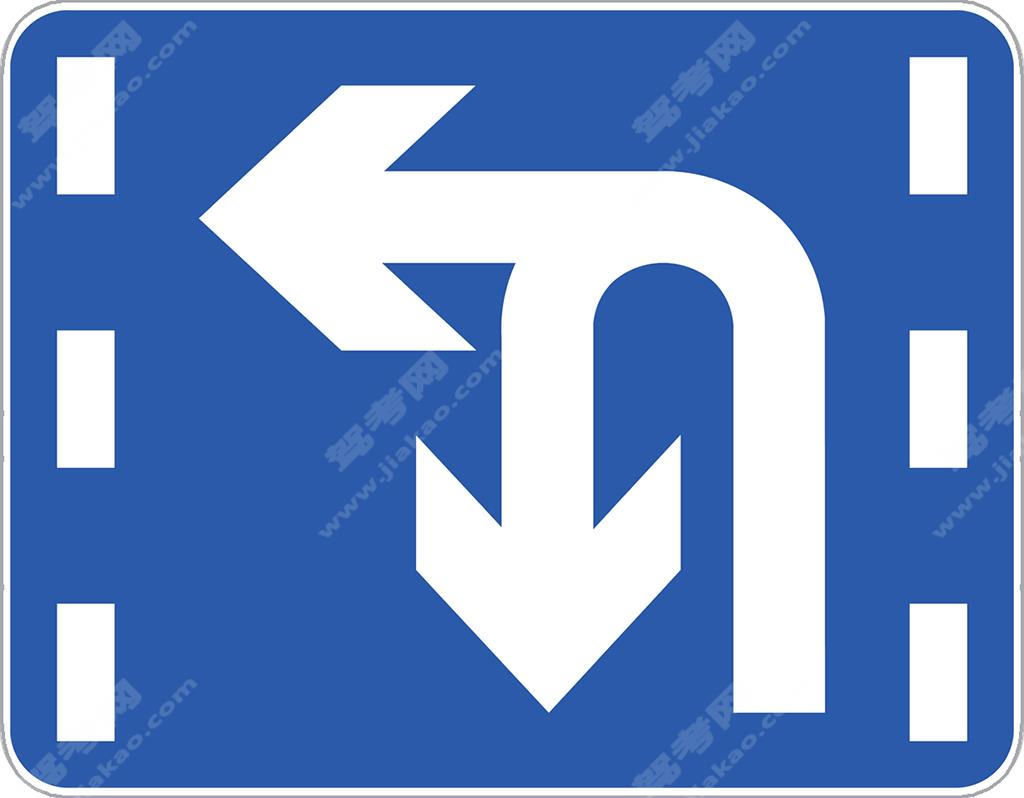掉头和左转合用车道

•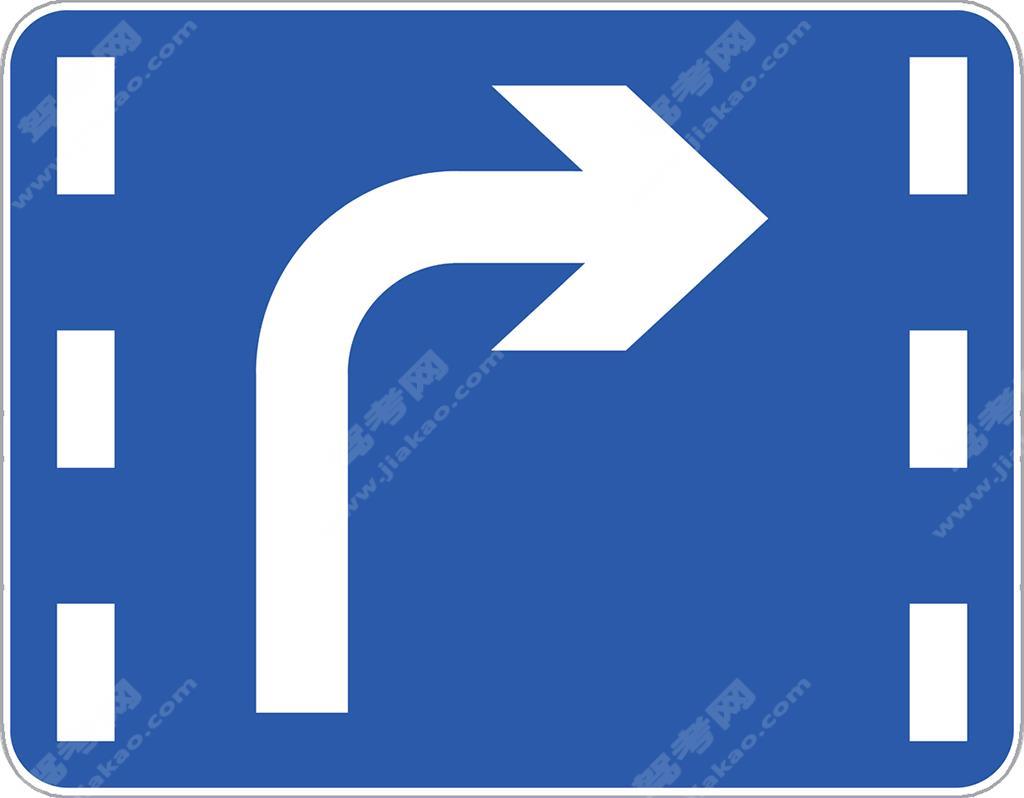右转车道

•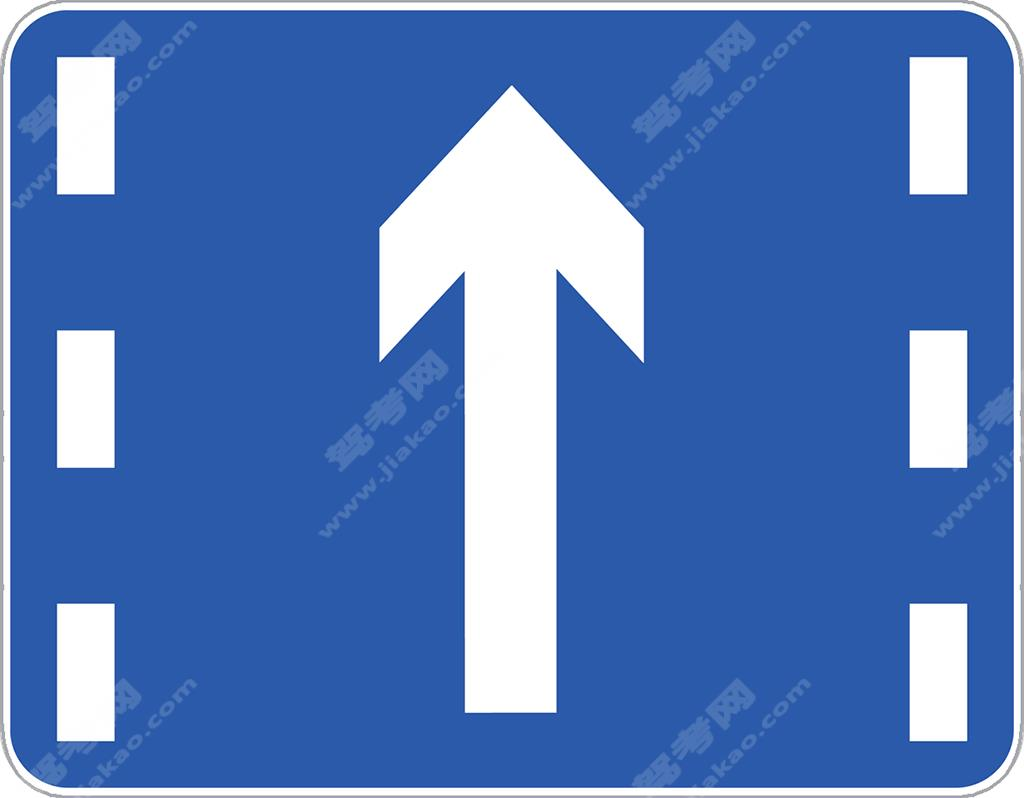直行车道

•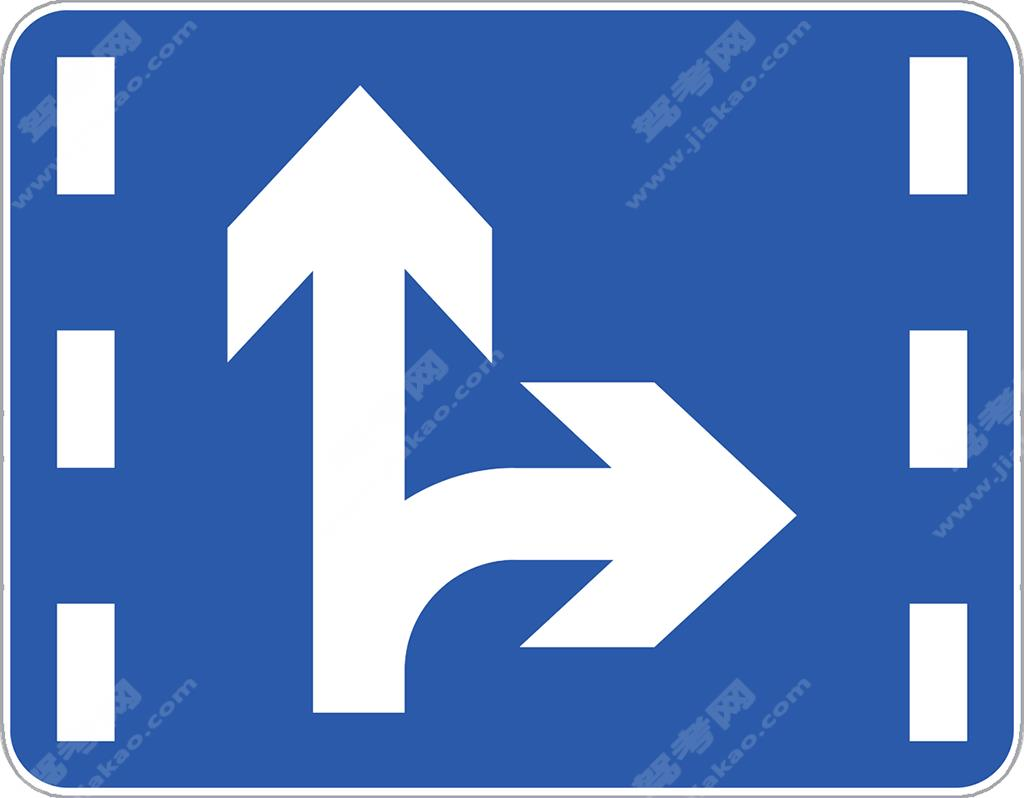直行和右转合用车道

•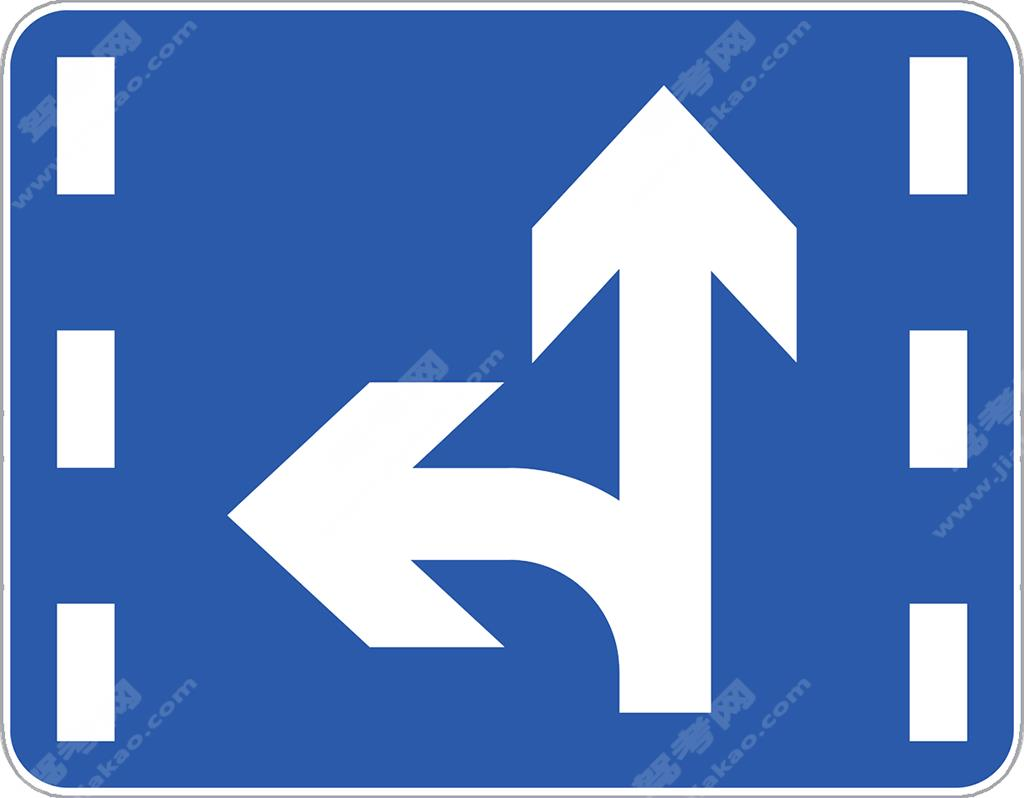直行和左转合用车道

•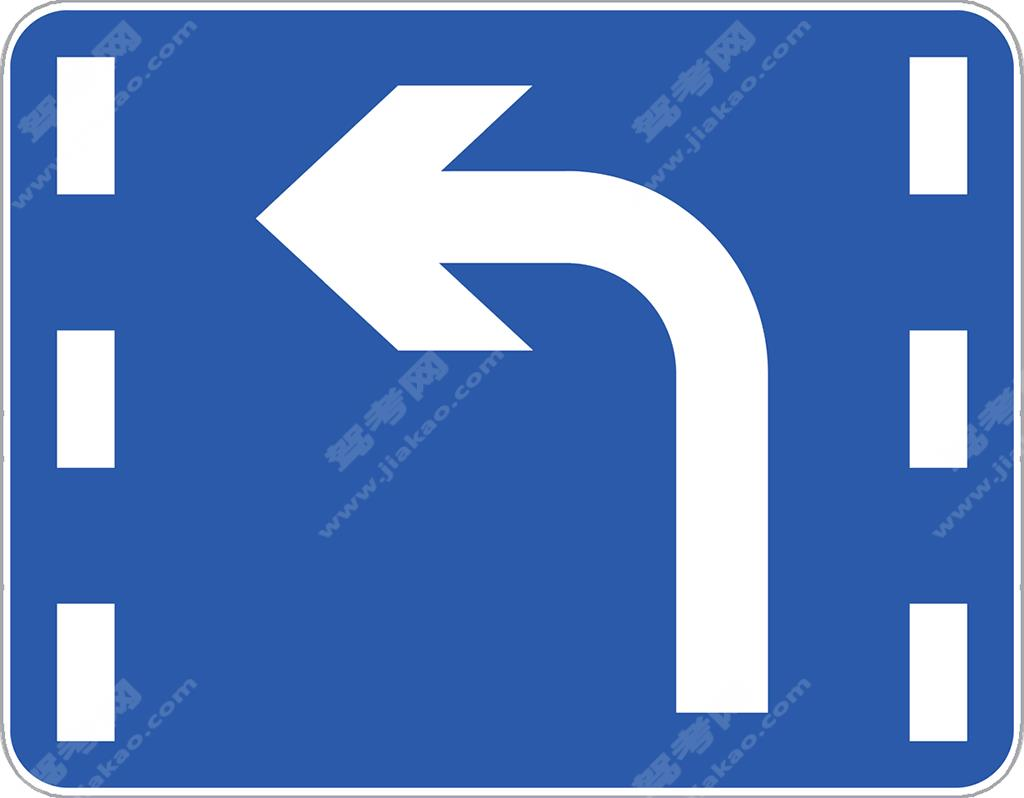左转车道

•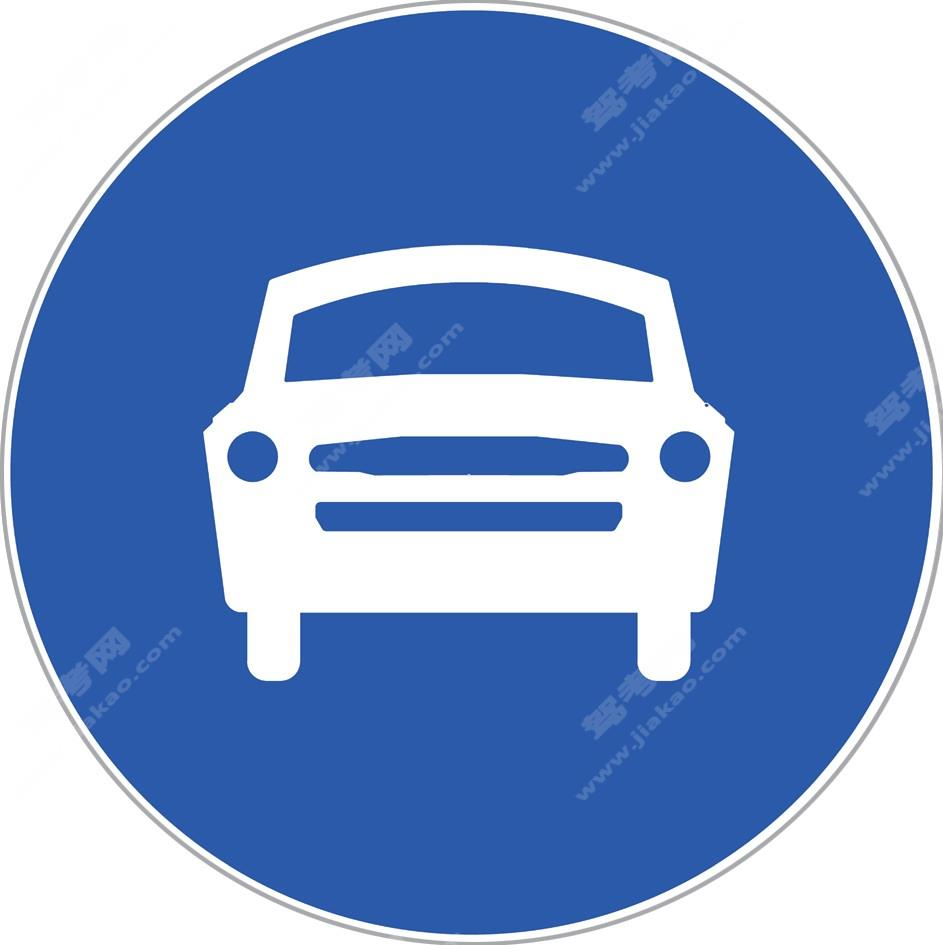机动车行驶

•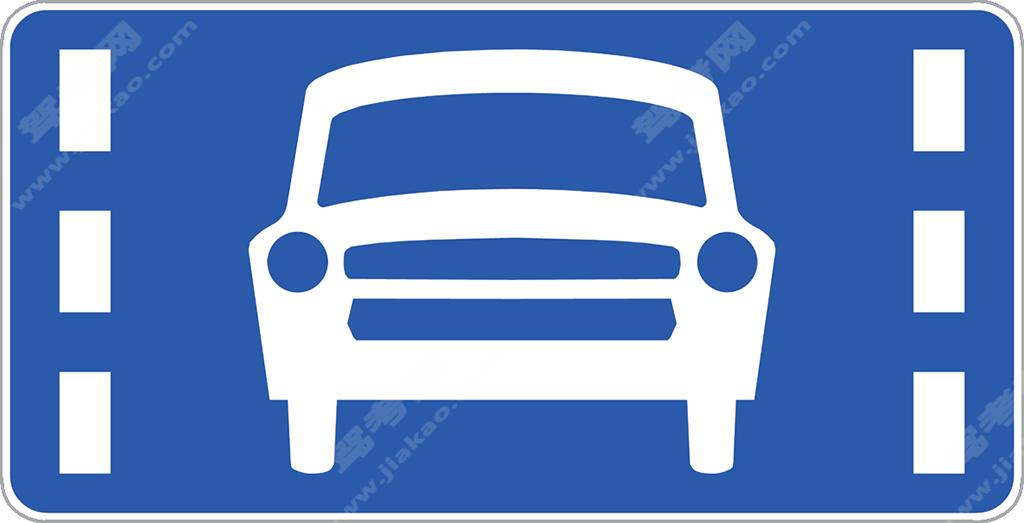机动车车道

•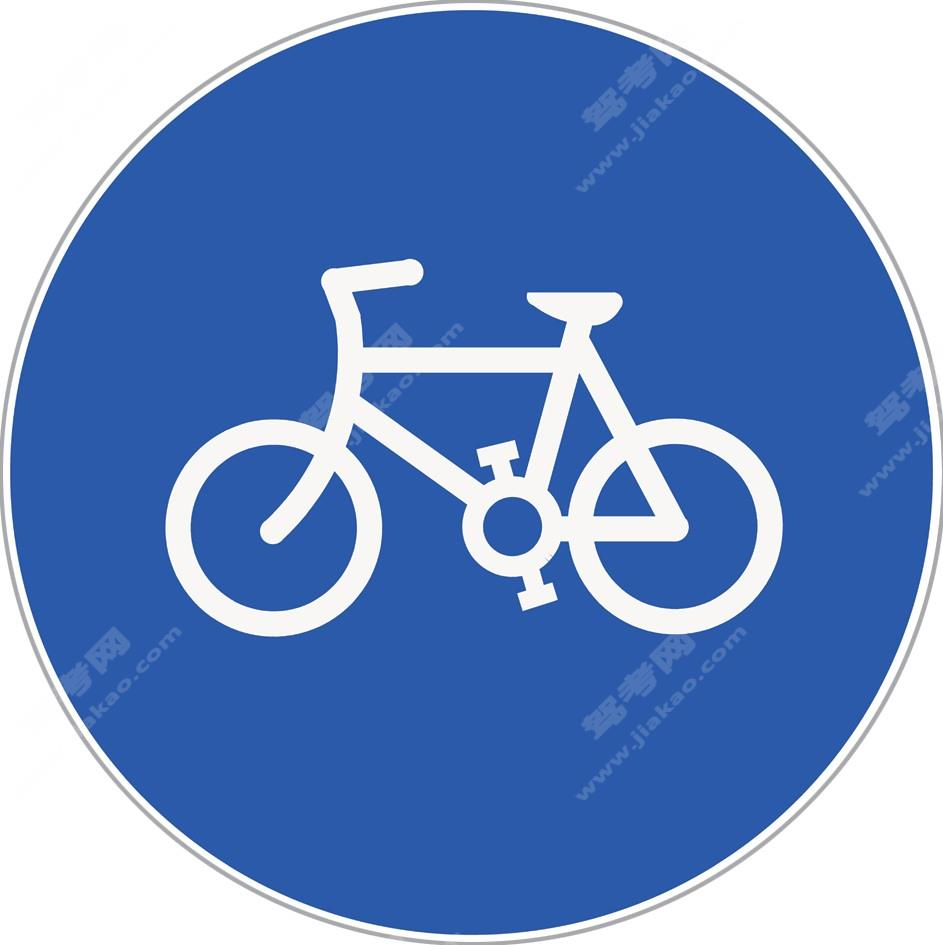非机动车行驶

•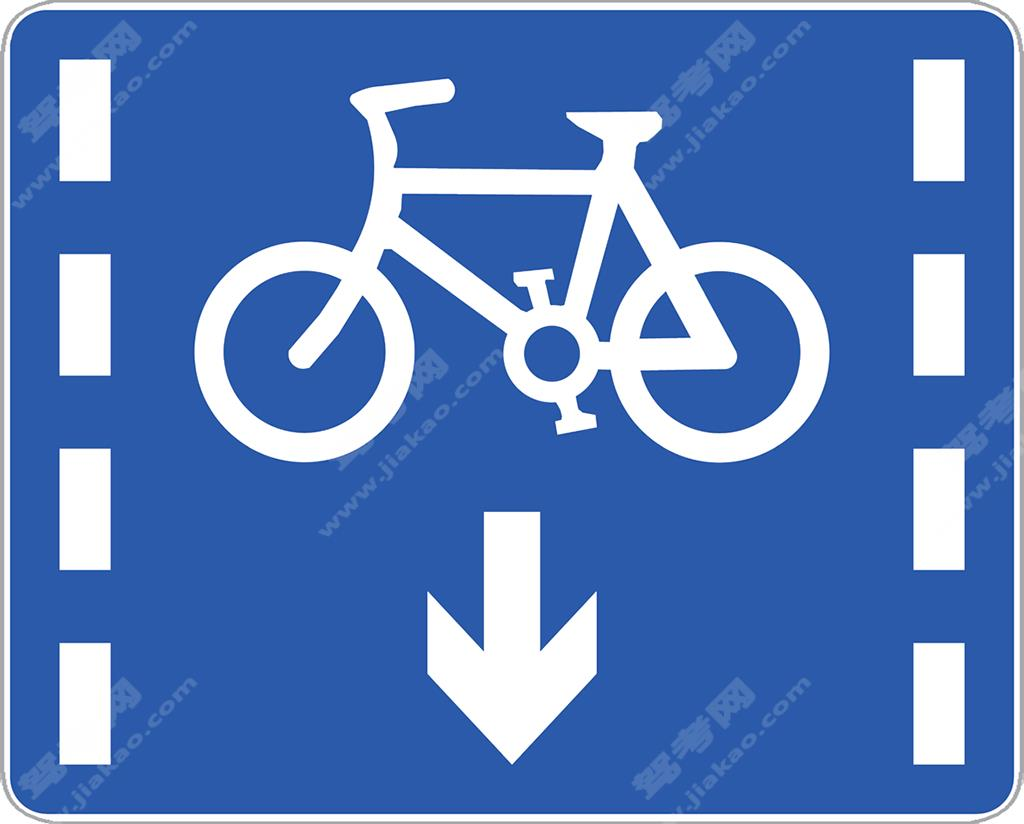非机动车车道

•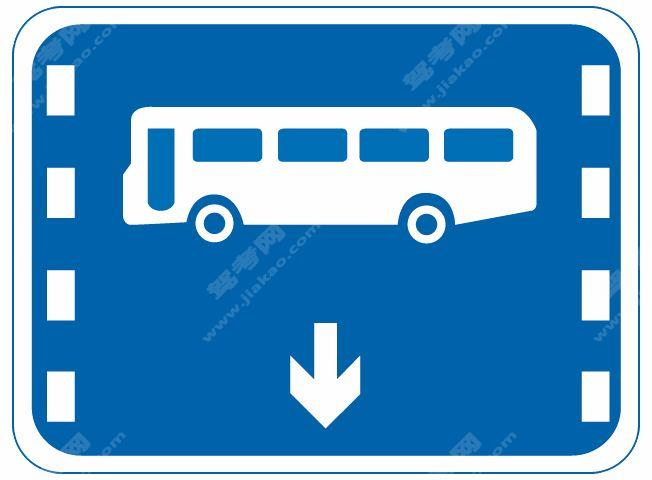公交线路专用车道（旧）

•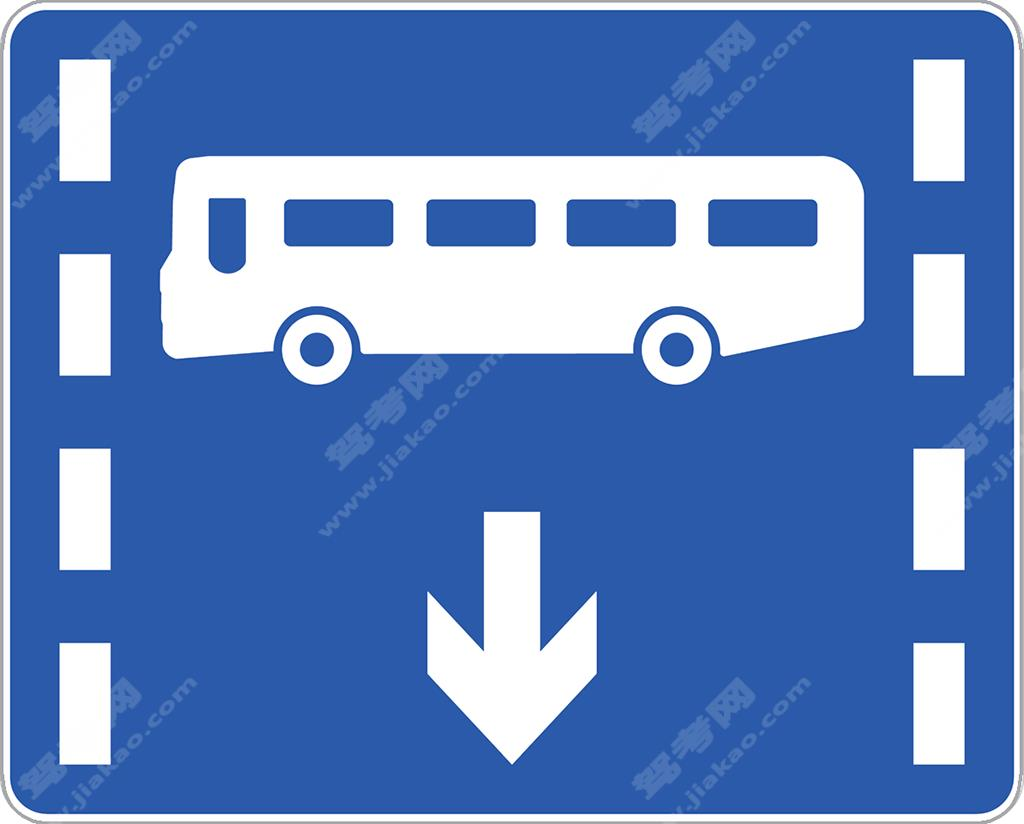公交专用车道（新）

•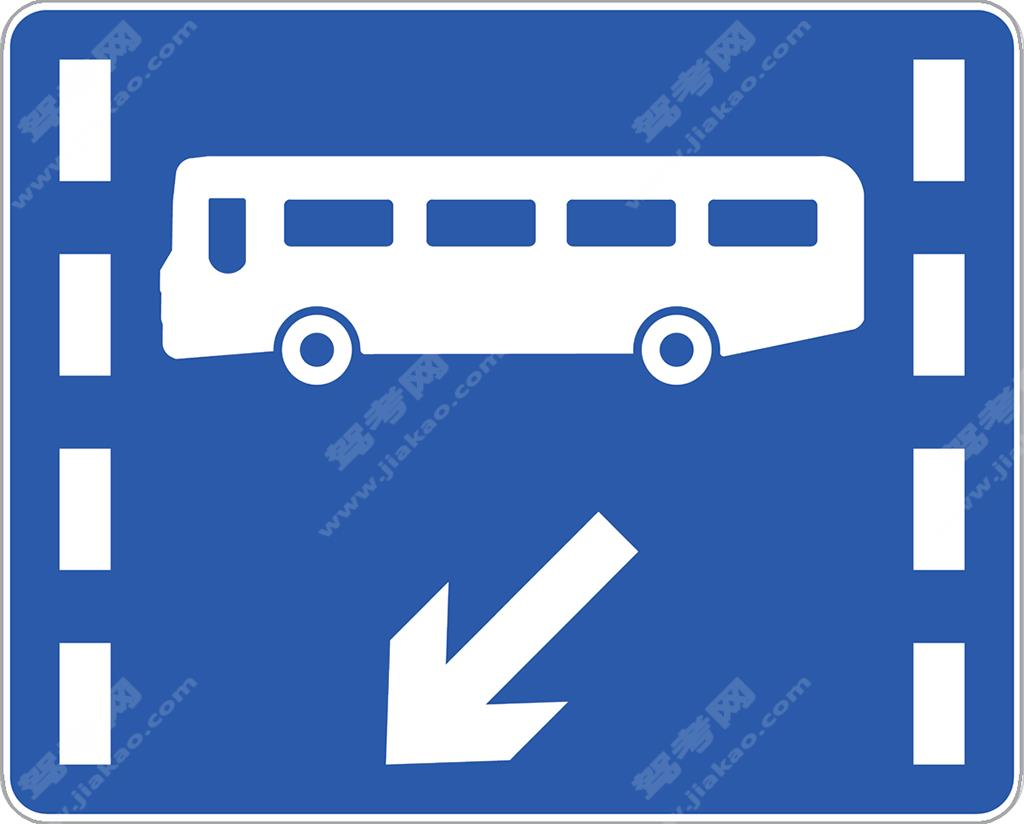公交专用车道（新）

•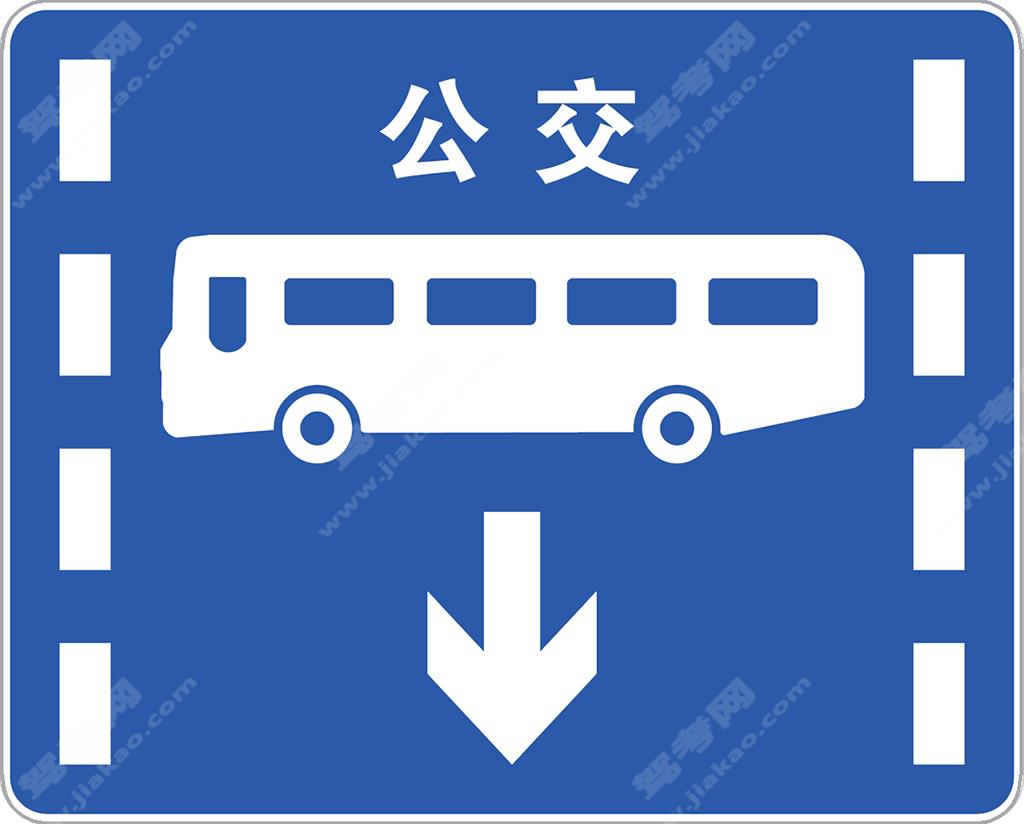公交专用车道（新）

•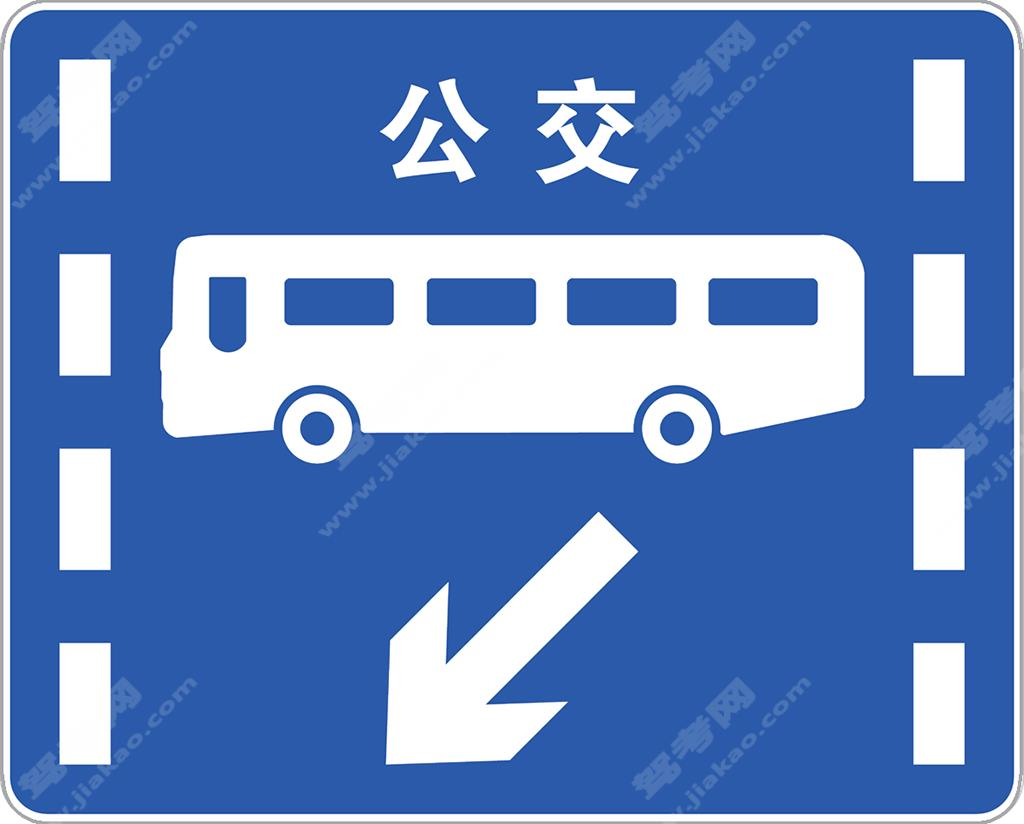公交专用车道（新）

•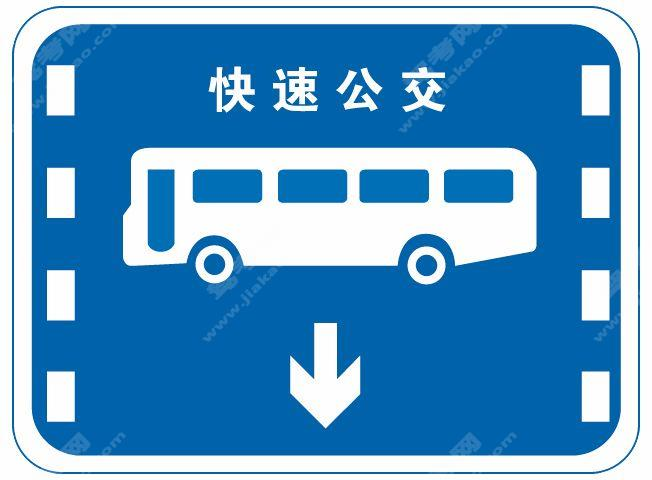快速公交系统专用车道（旧）

•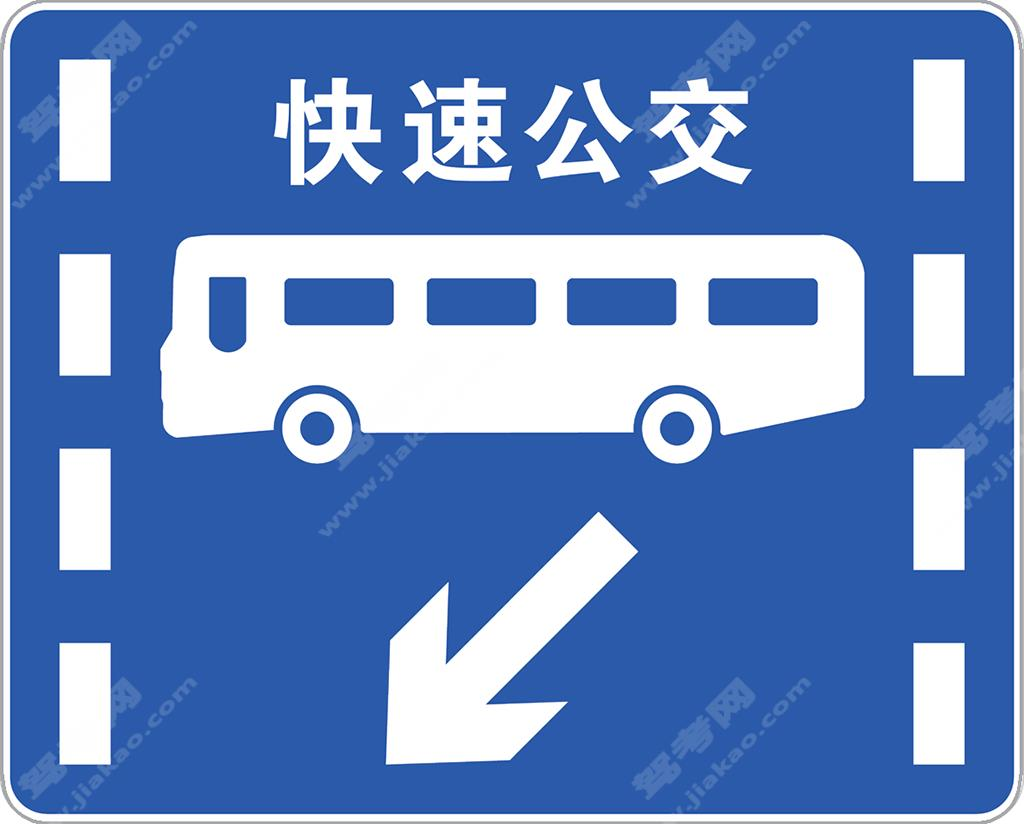快速公交系统专用车道（新）

•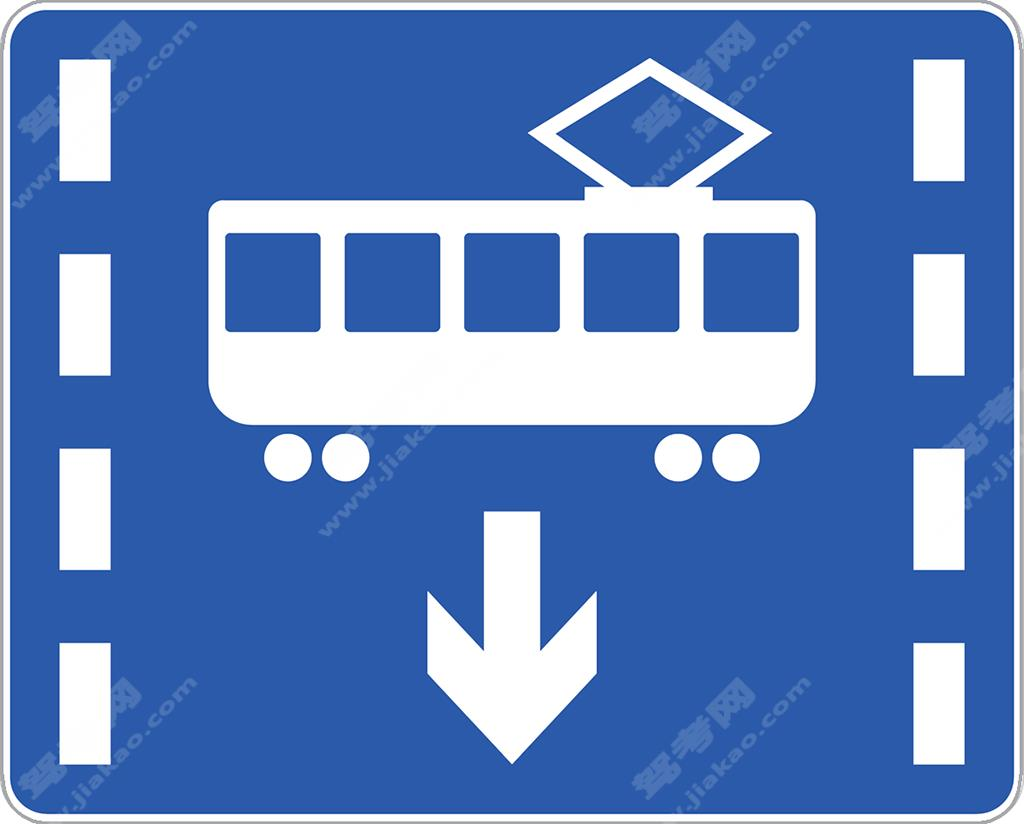有轨电车专用车道（新）

•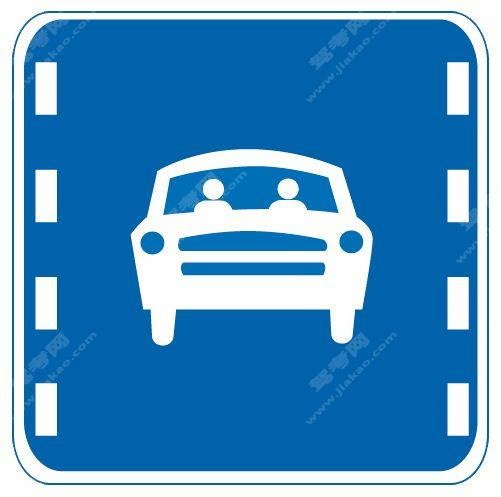多乘员车辆专用车道（旧）

•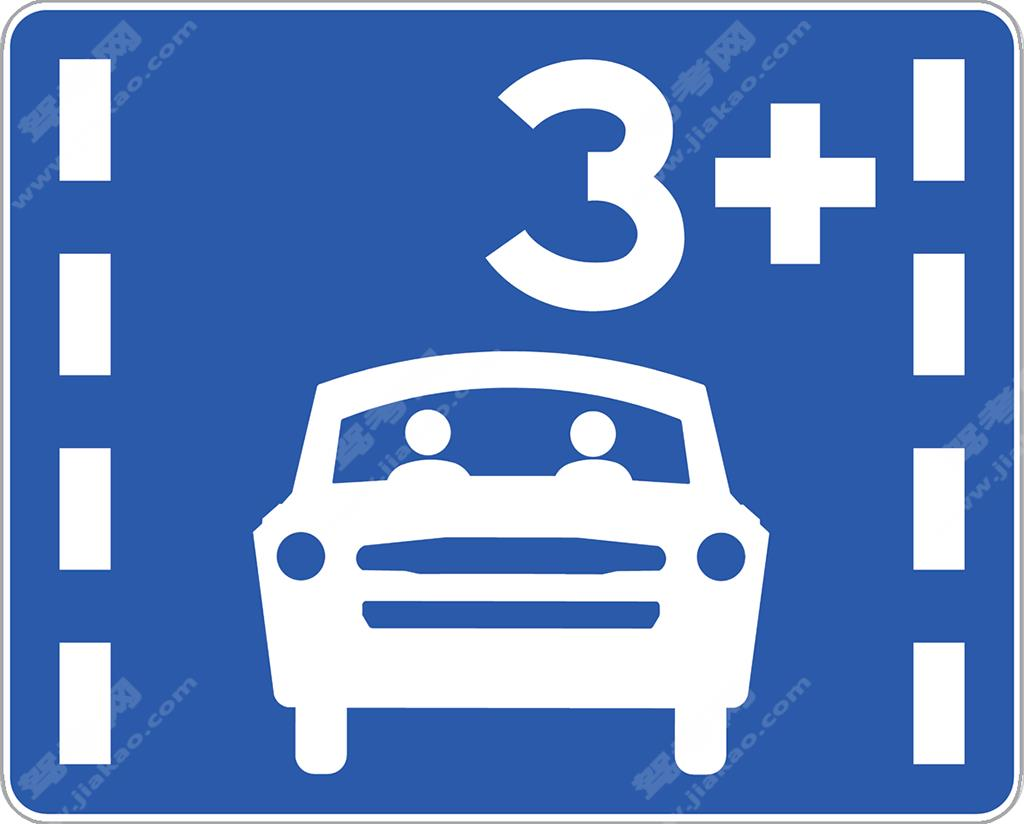多乘员车辆专用车道

•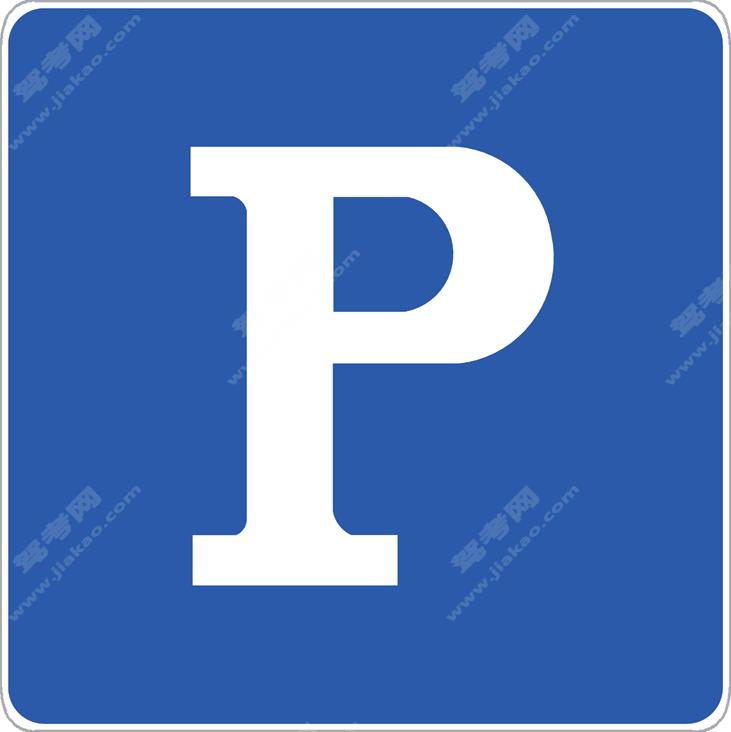停车位

•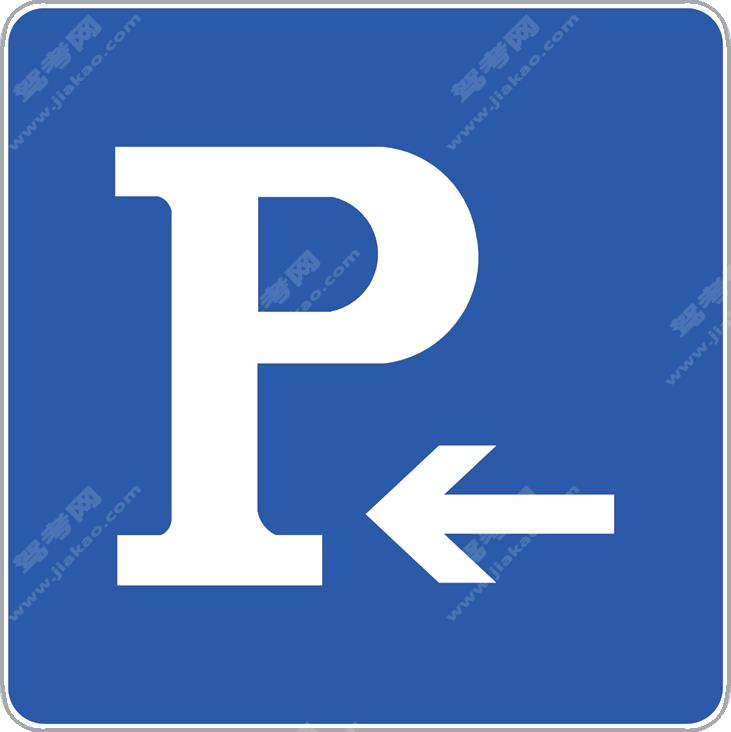停车位

•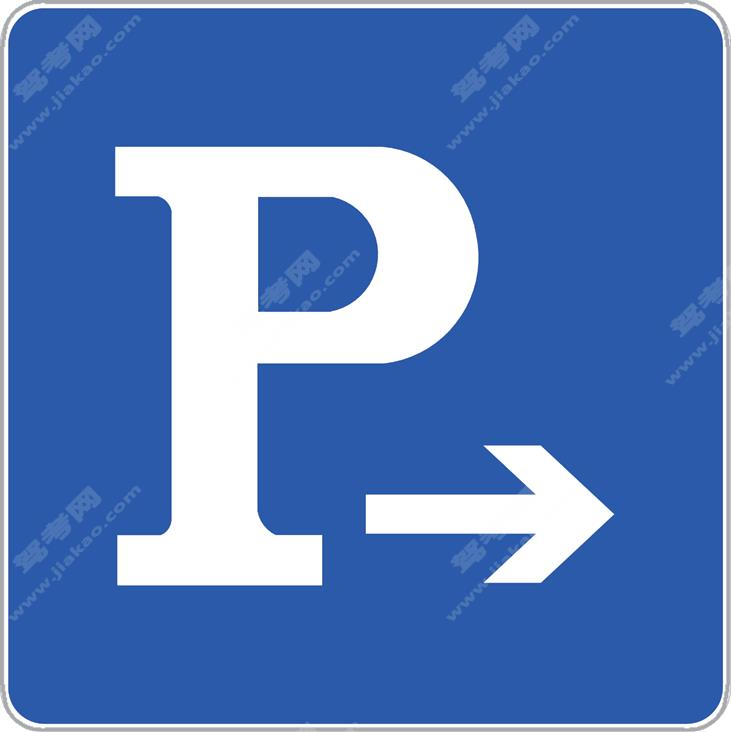停车位

•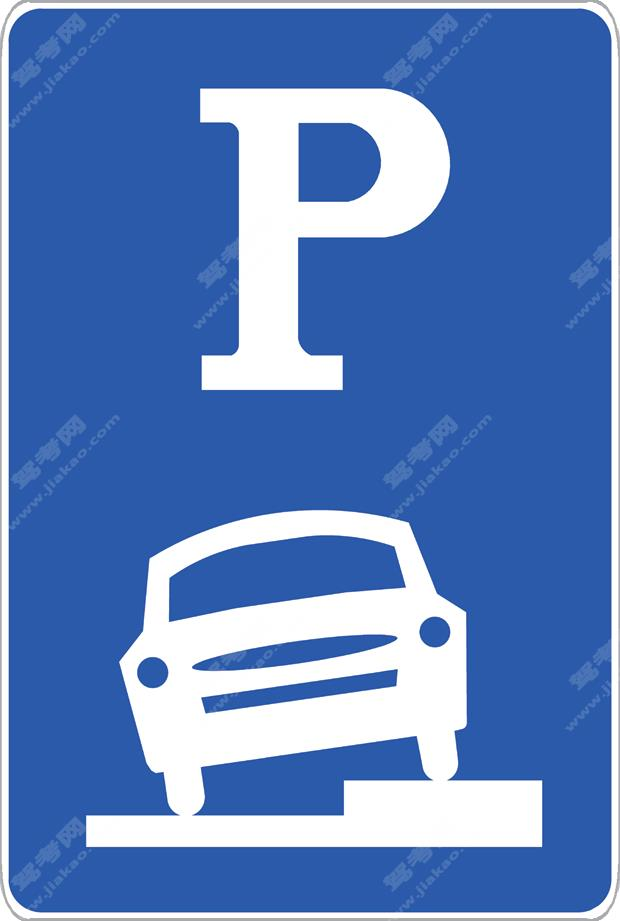停车位

•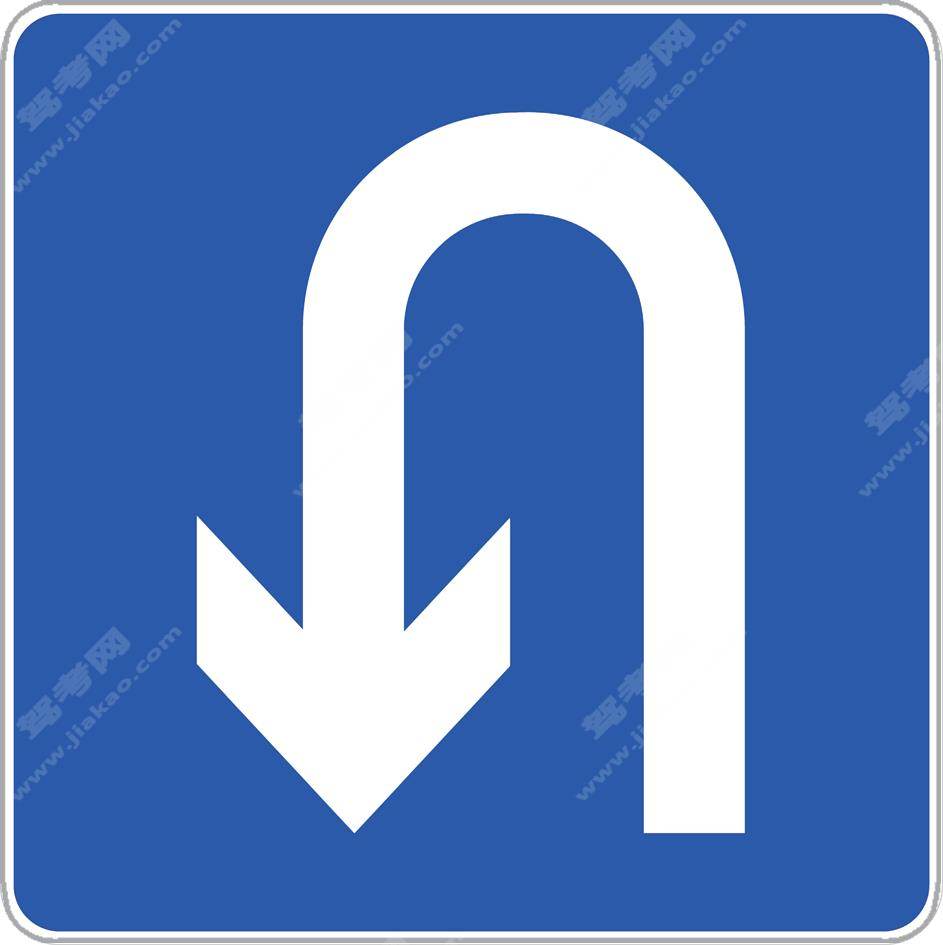允许掉头

•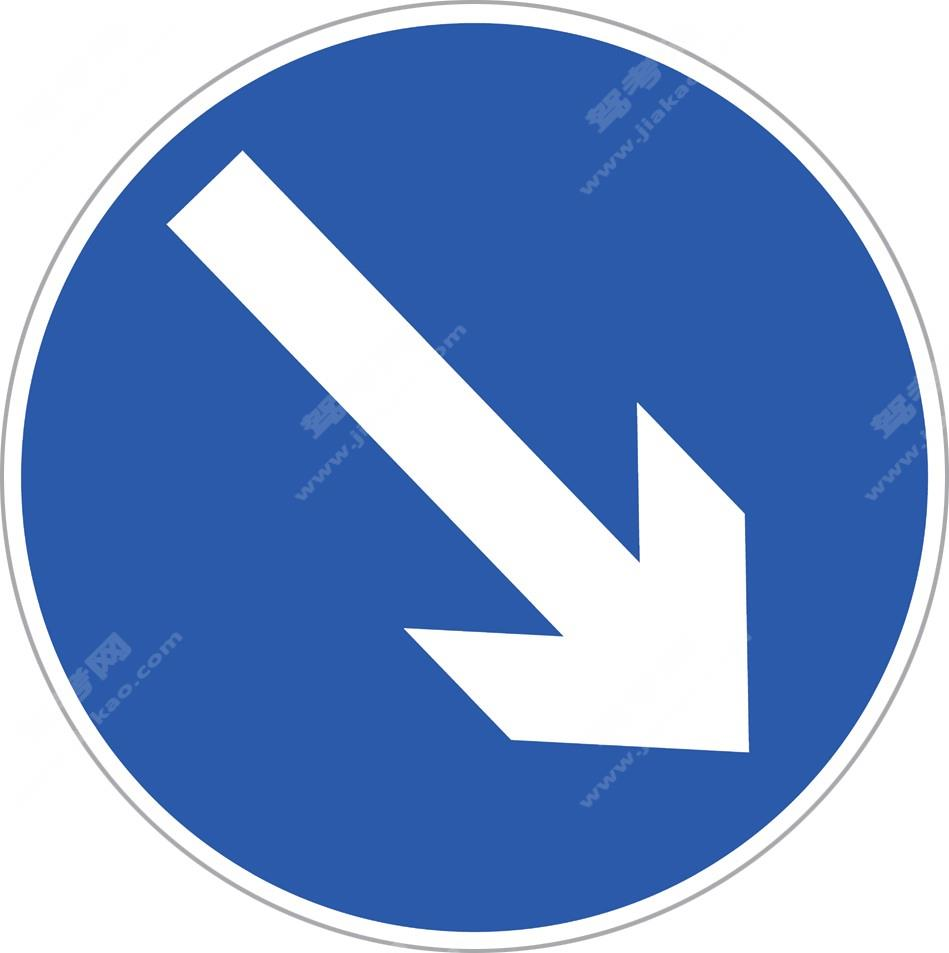分隔带右侧行驶（新）

•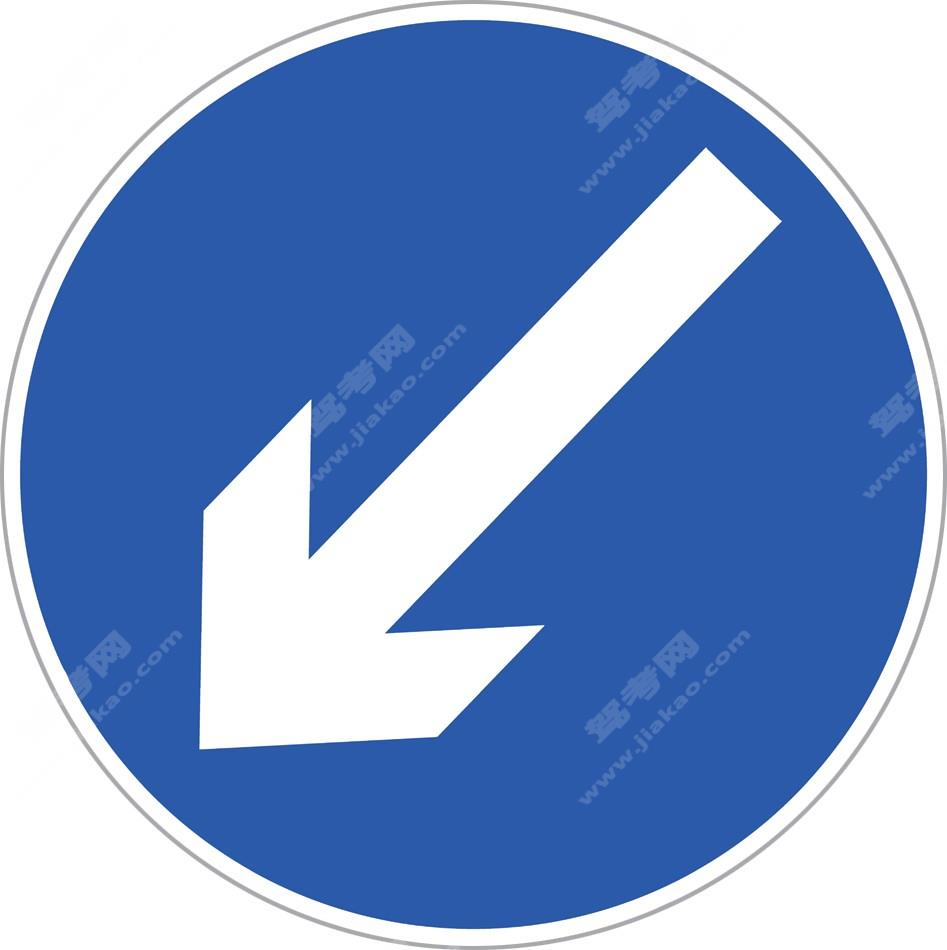分隔带左侧行驶（新）

•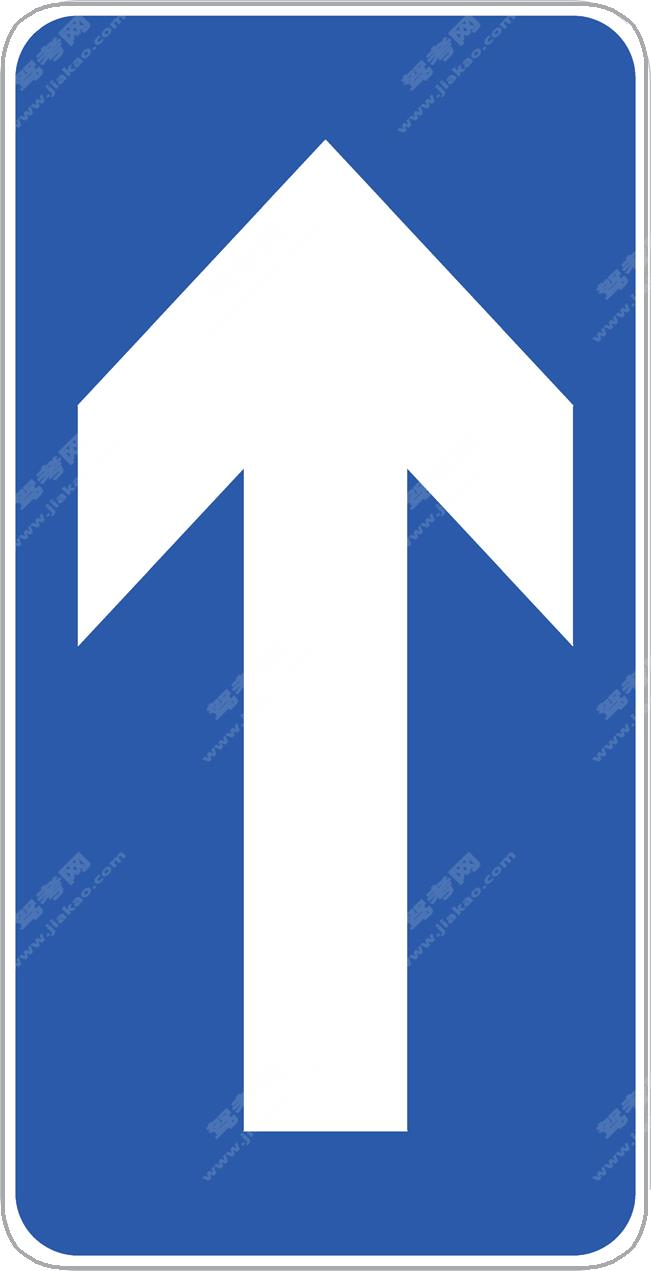单行路(直行)（旧）

•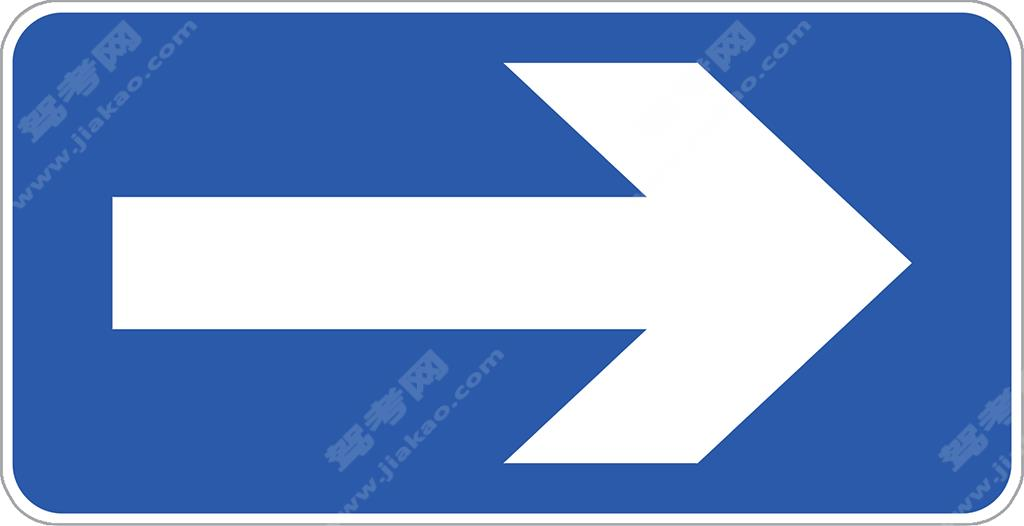单行路(向左或向右)（旧）

•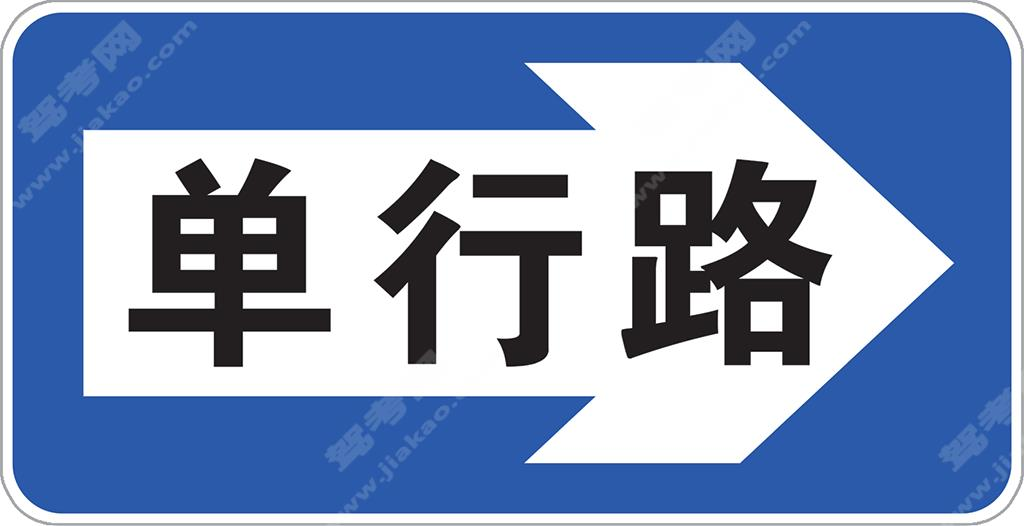单行路（向右）（新）

•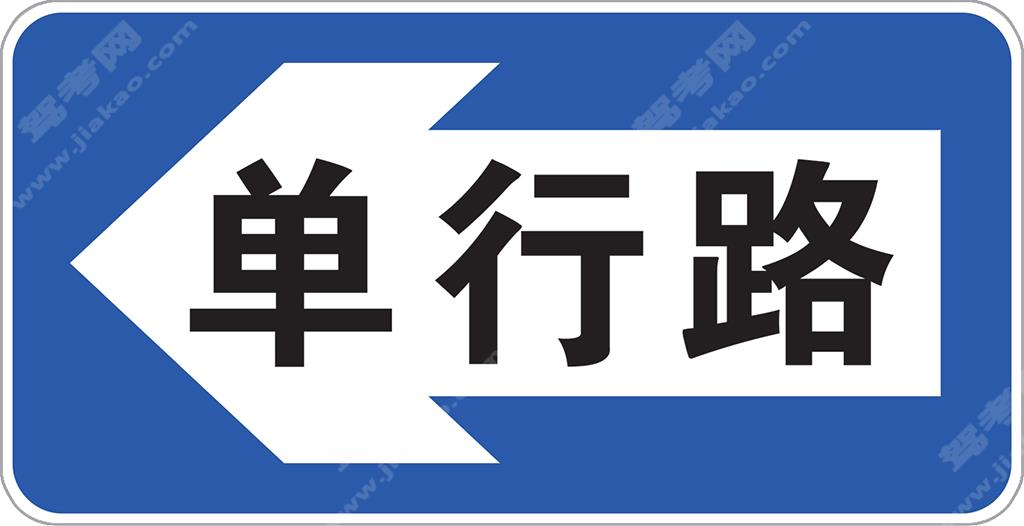单行路（向左）（新）

•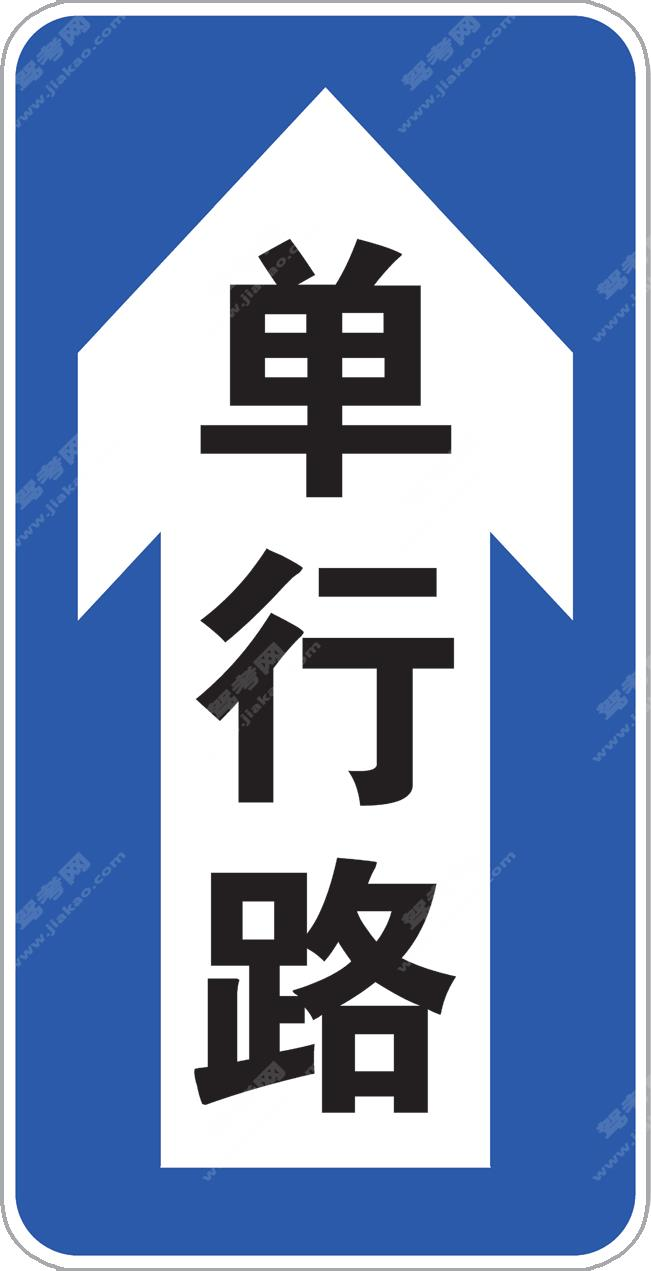单行路（直行）（新）

•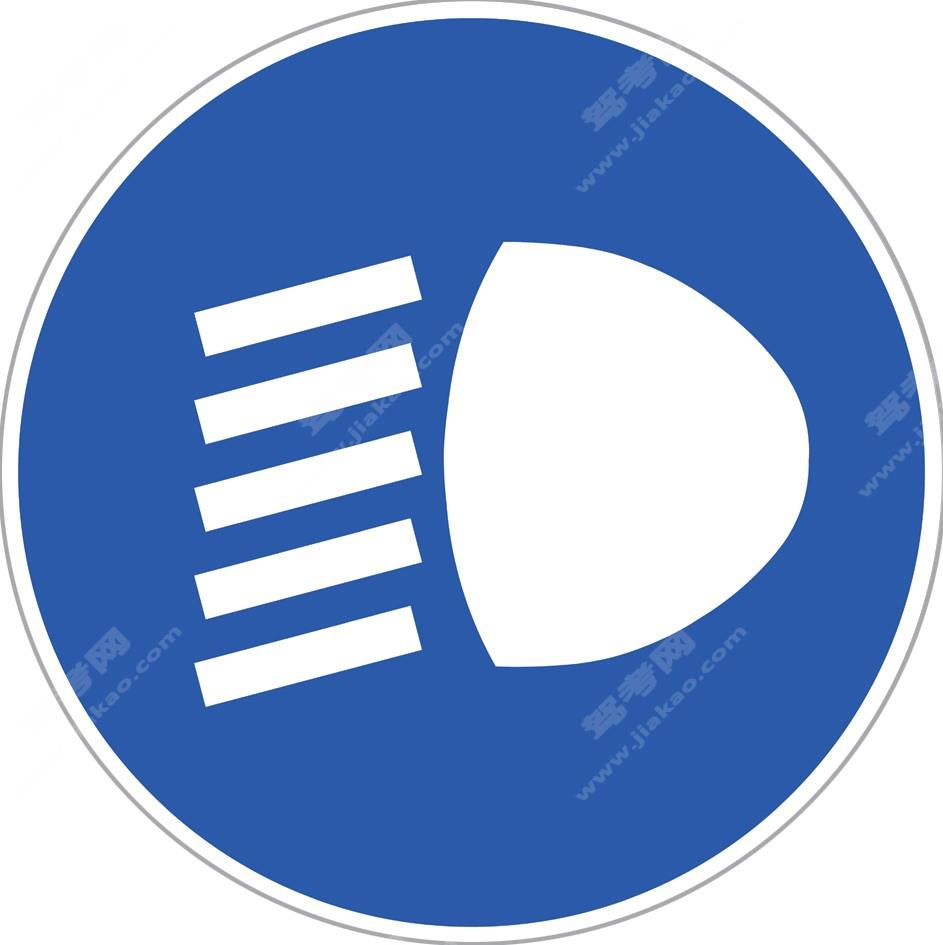开车灯（新）

•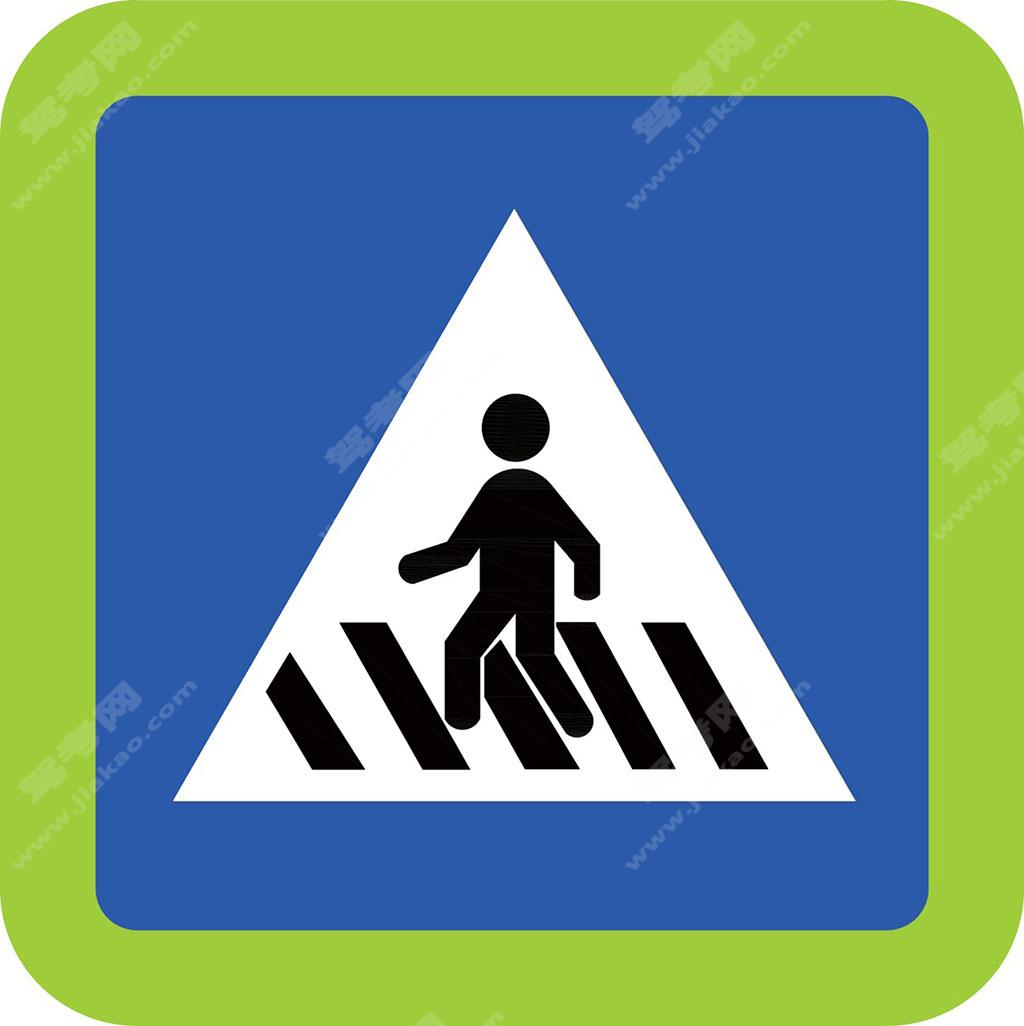人行横道（新）

•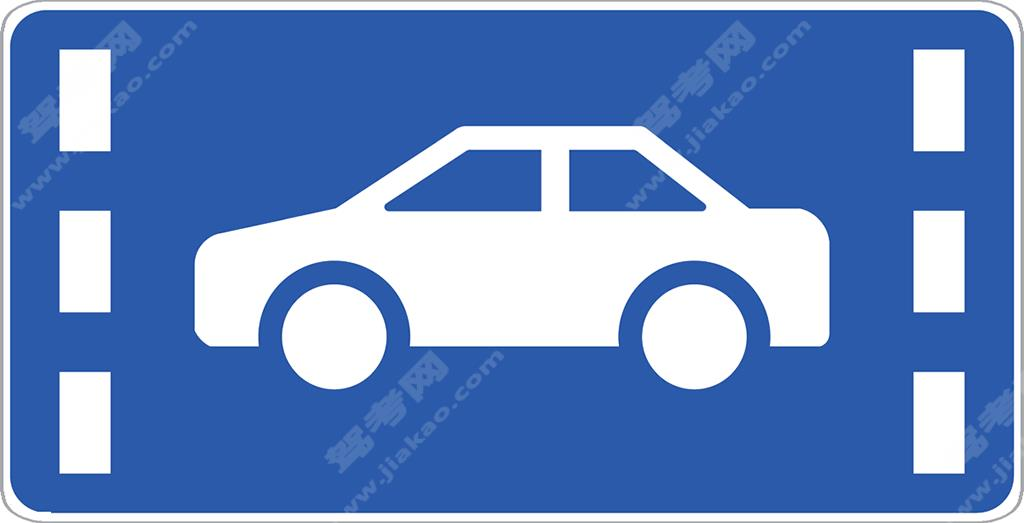小型客车车道（新）

•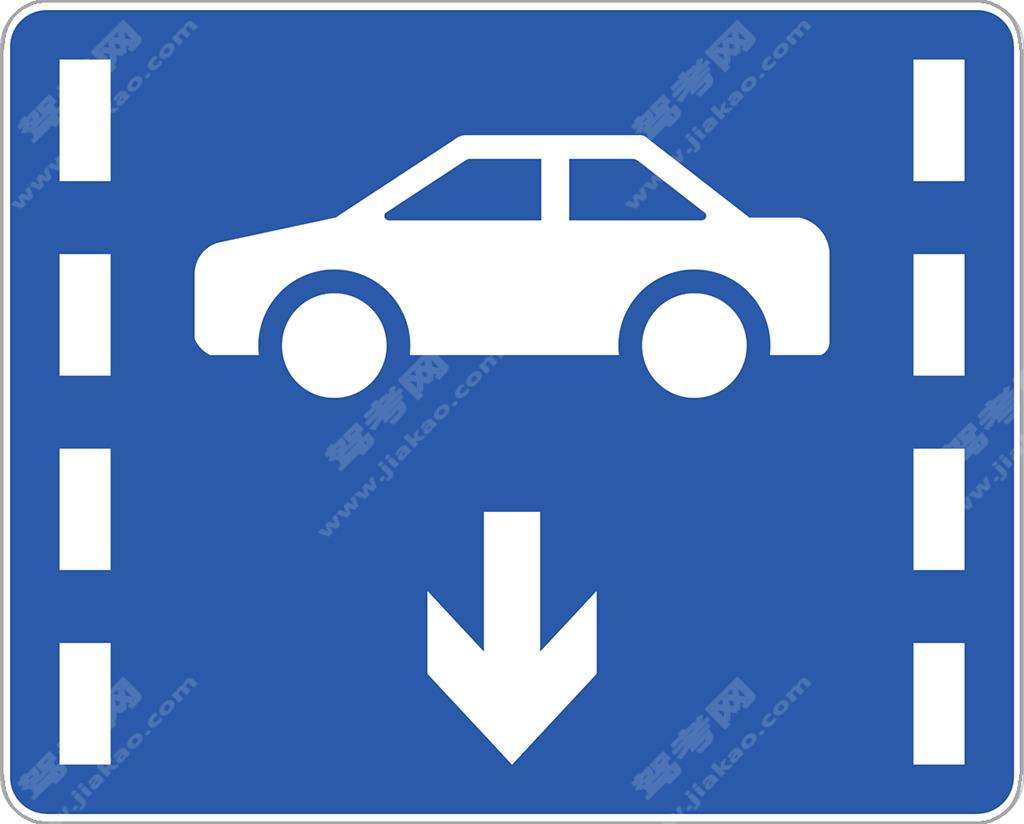小型客车车道（新）

•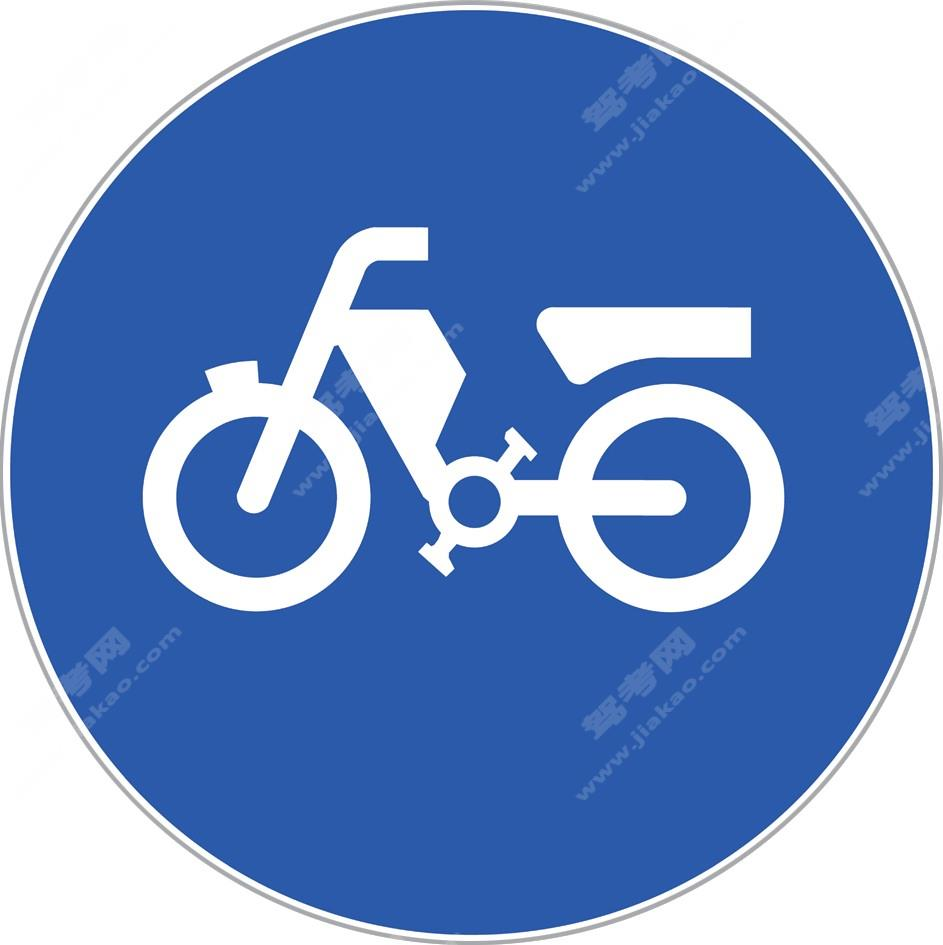电动自行车行驶（新）

•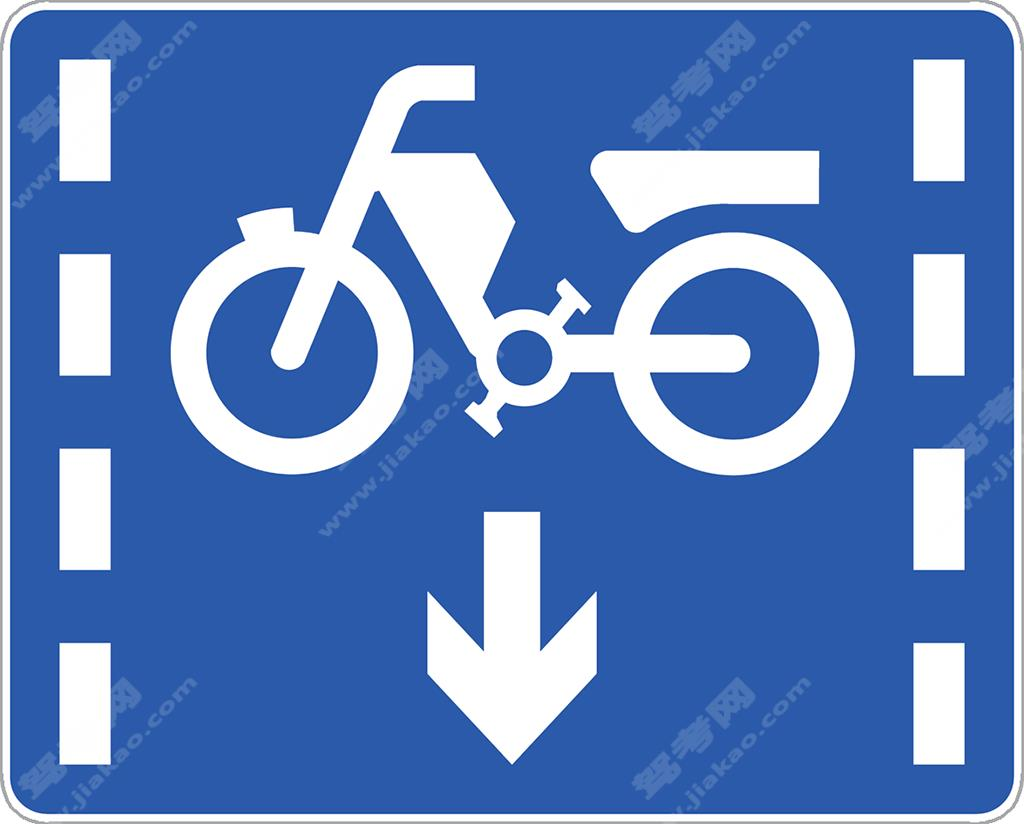电动自行车车道（新）

•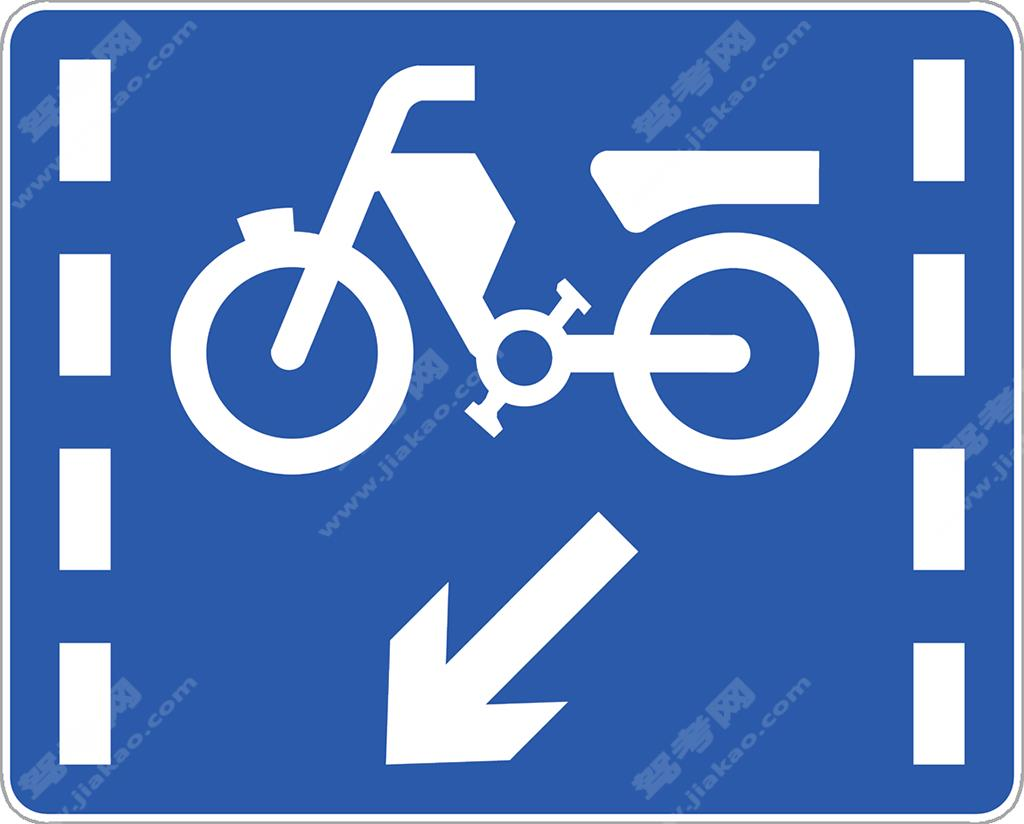电动自行车车道（新）

•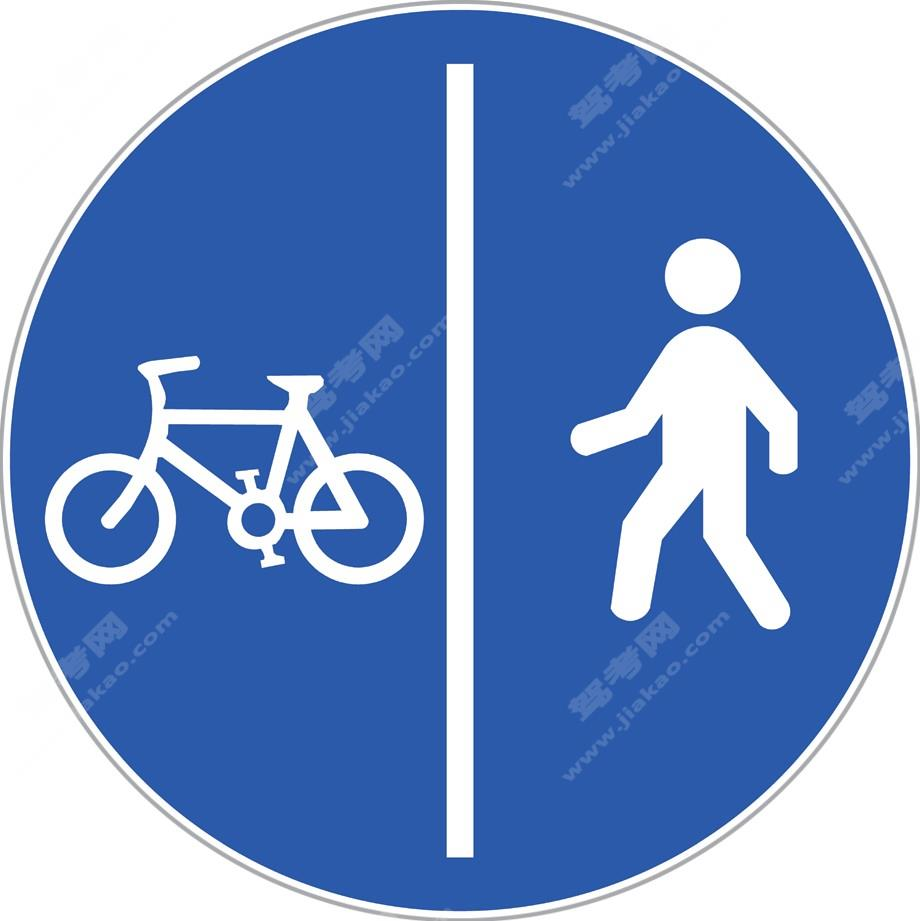非机动车与行人分开空间通行（新）

•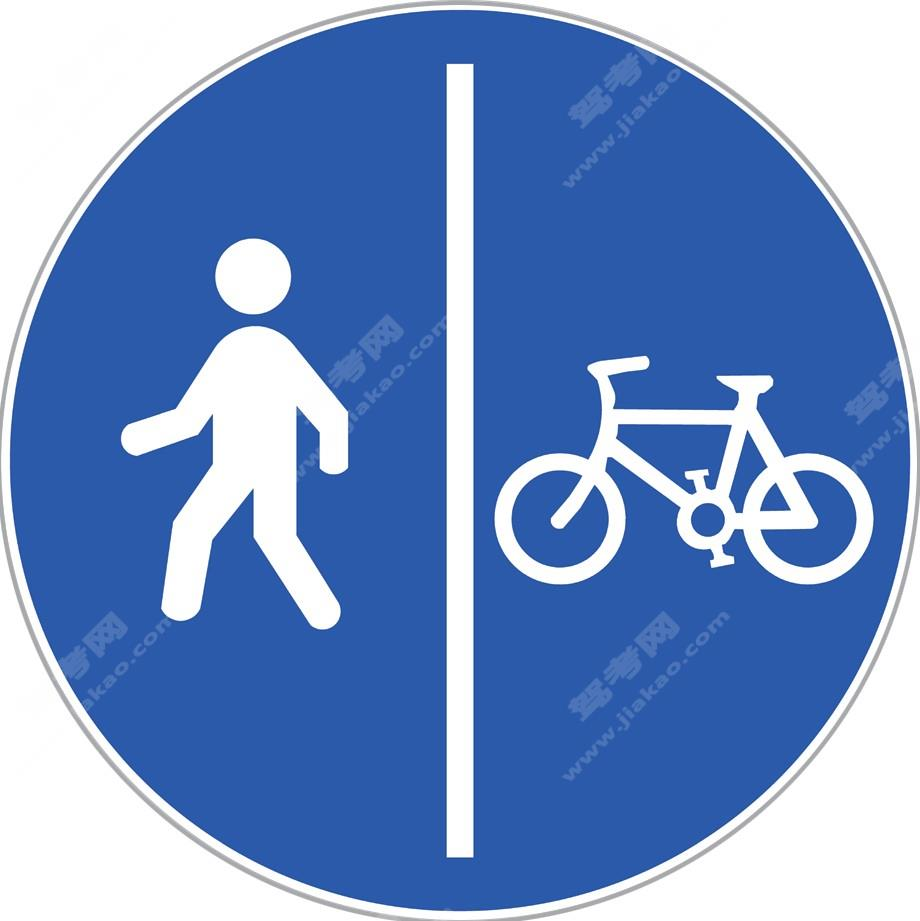非机动车与行人分开空间通行（新）

•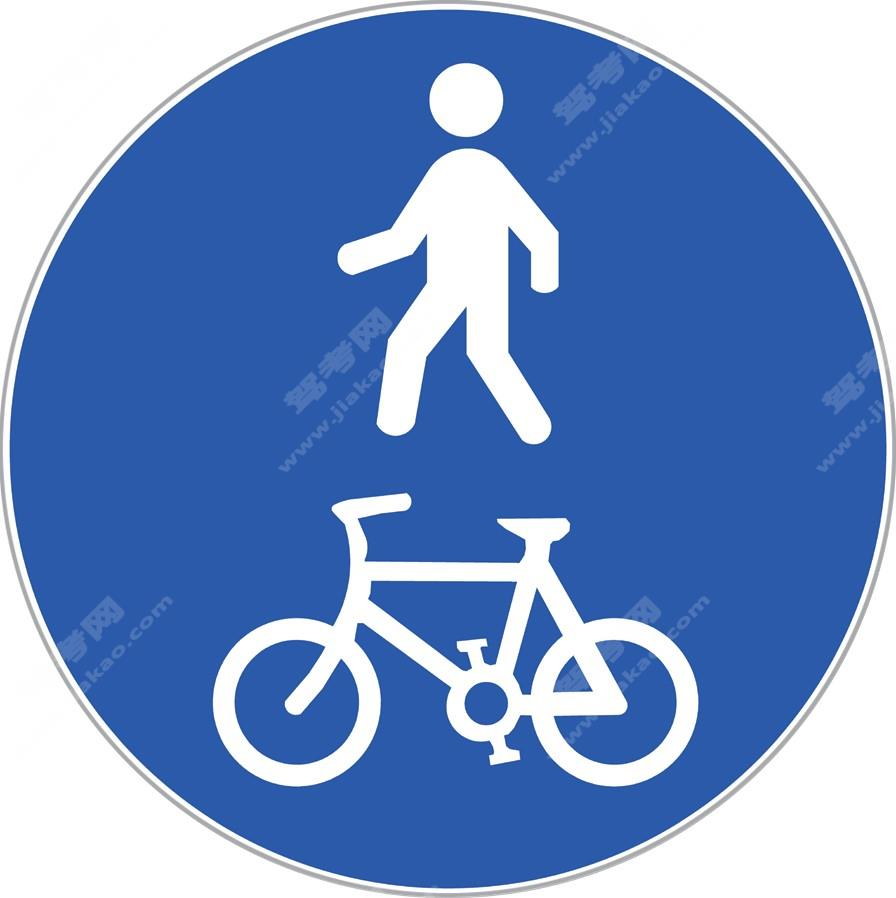非机动车与行人共享空间通行（新）

•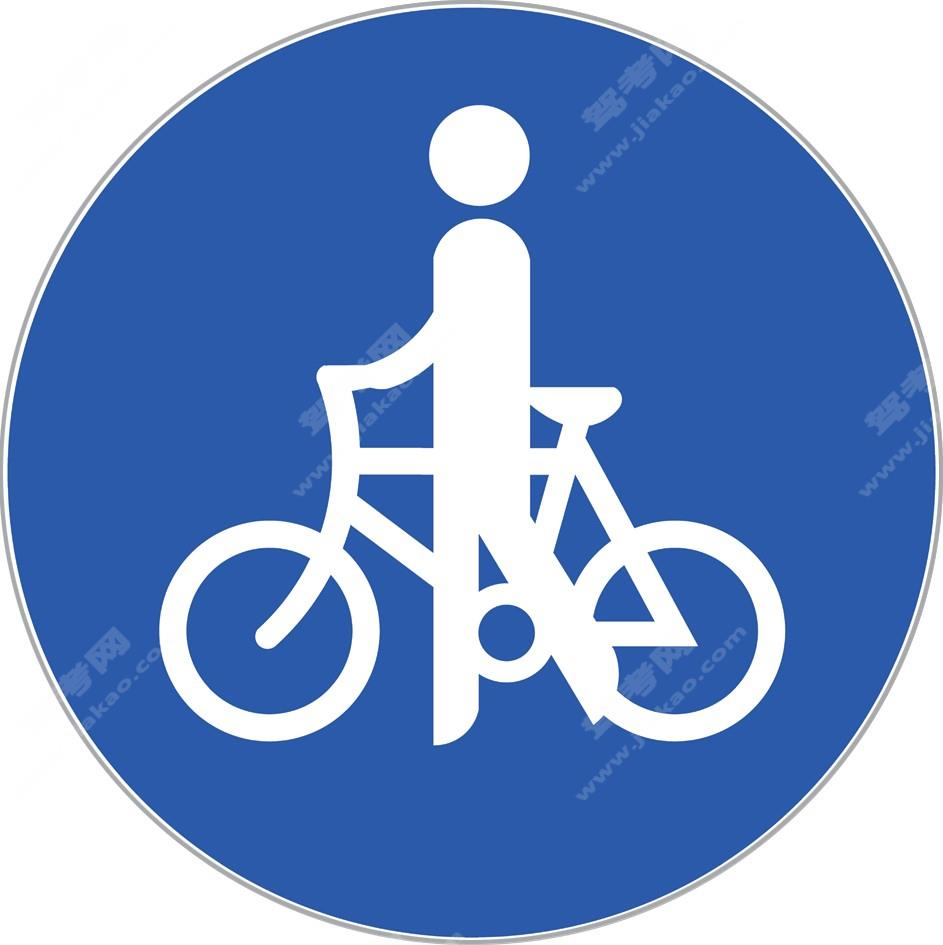非机动车推行（新）

•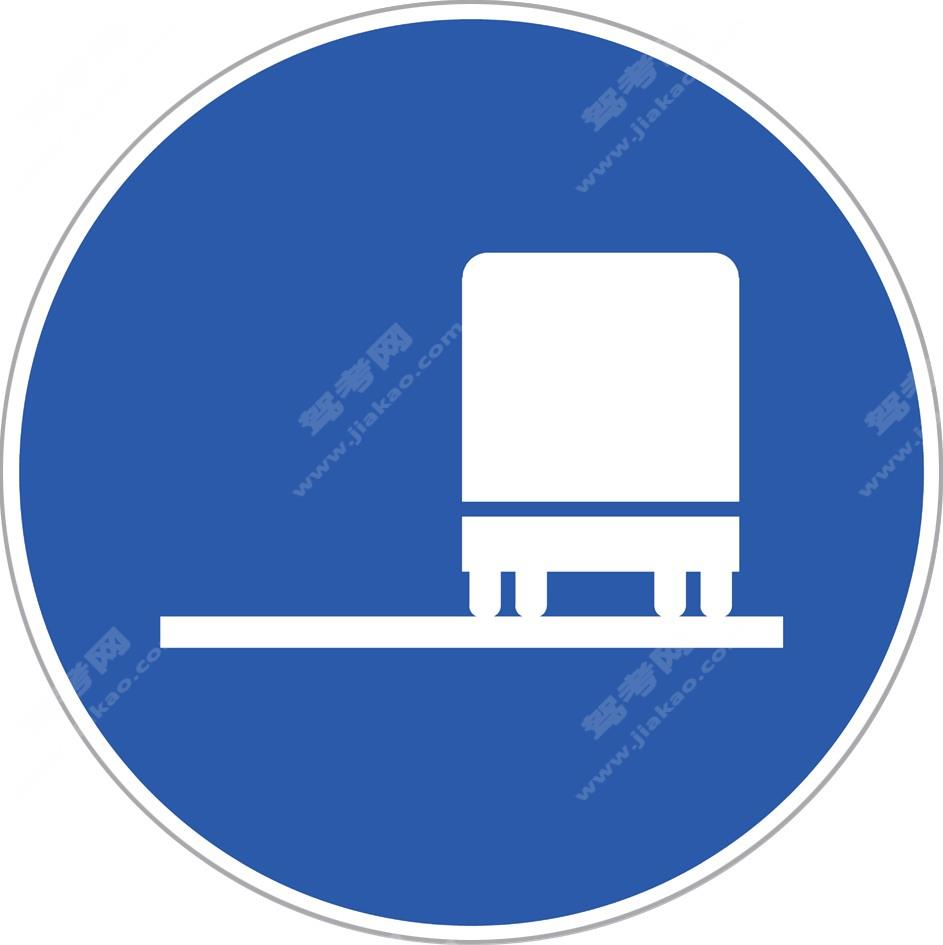靠右侧车道行驶（新）

•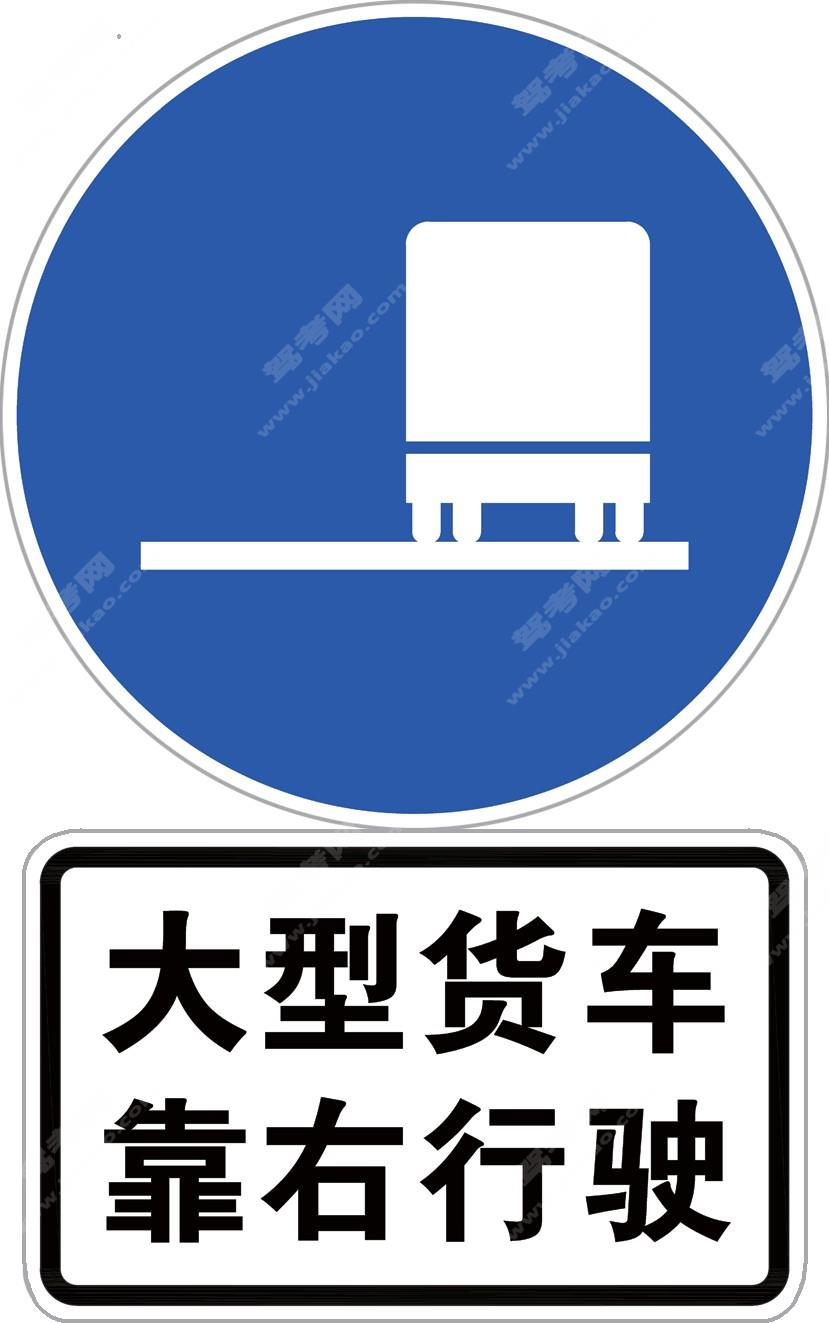靠右侧车道行驶标志加辅助标志（新）

•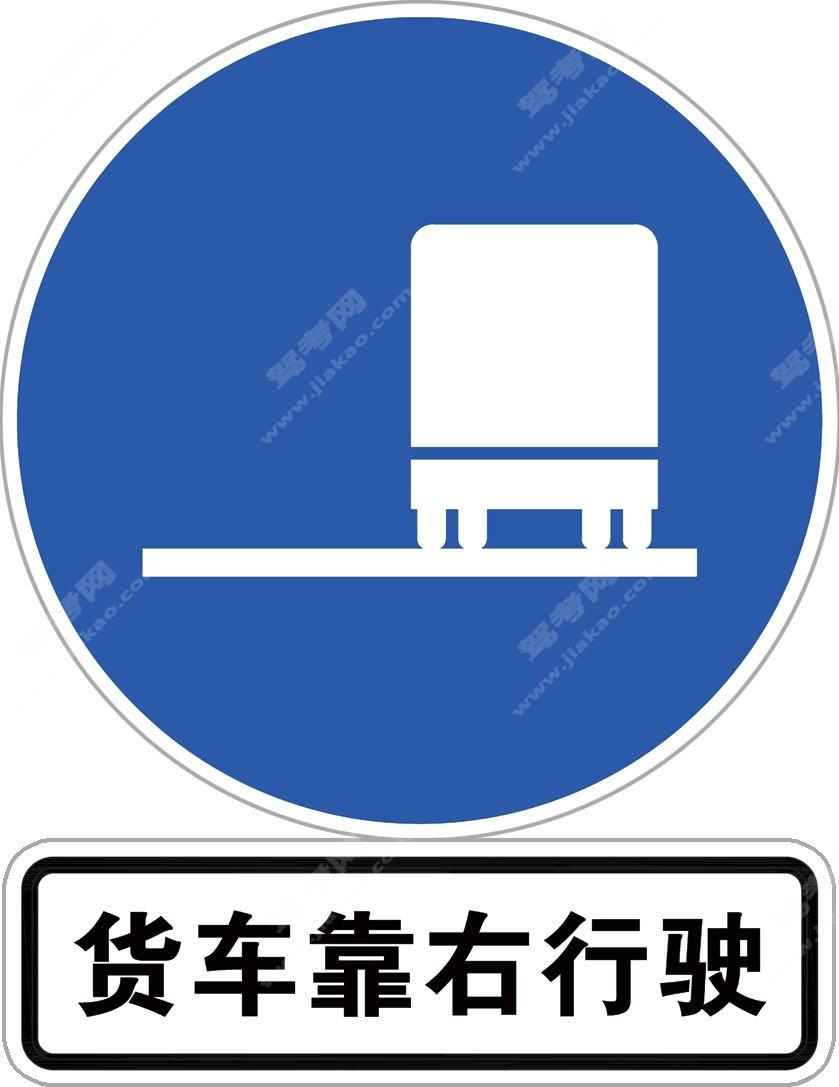靠右侧车道行驶标志加辅助标志（新）

•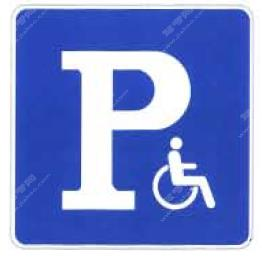残疾人专用停车位（旧）

•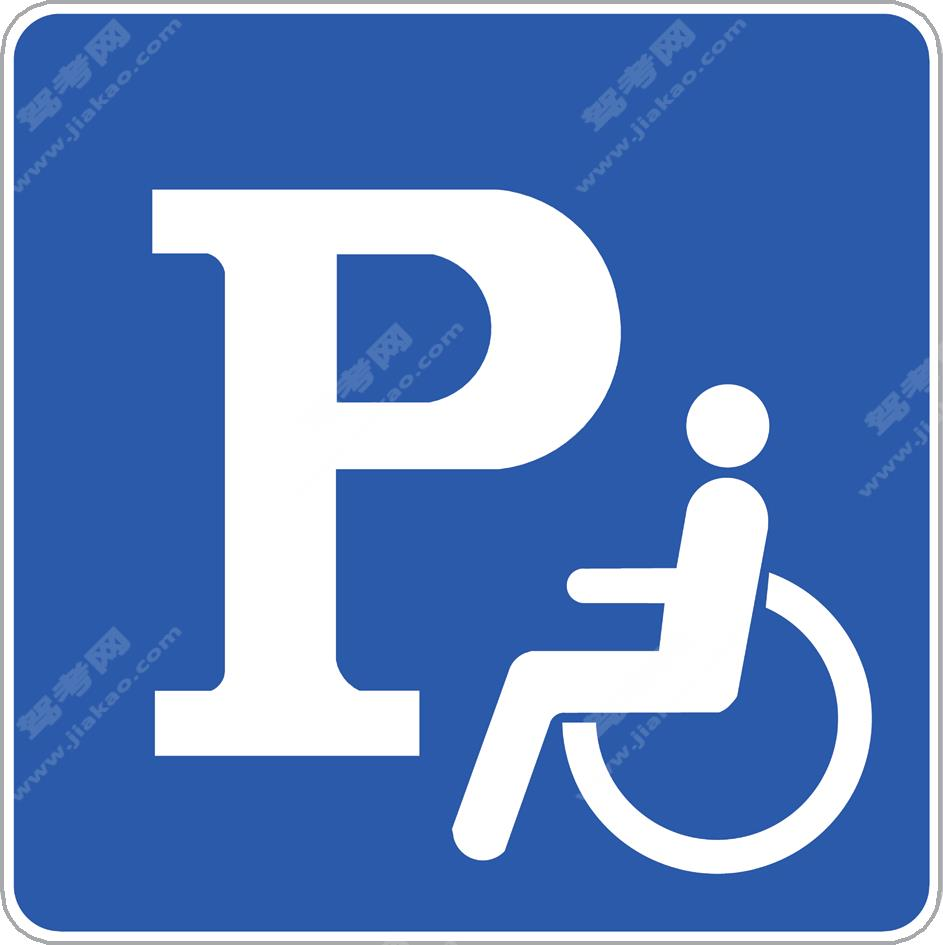残疾人专用停车位（新）

•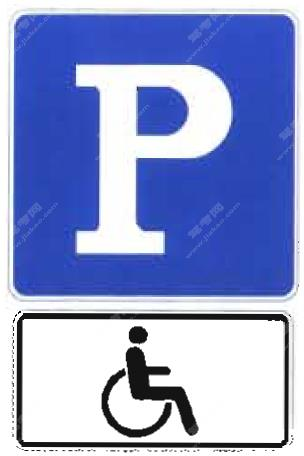残疾人专用停车位（旧）

•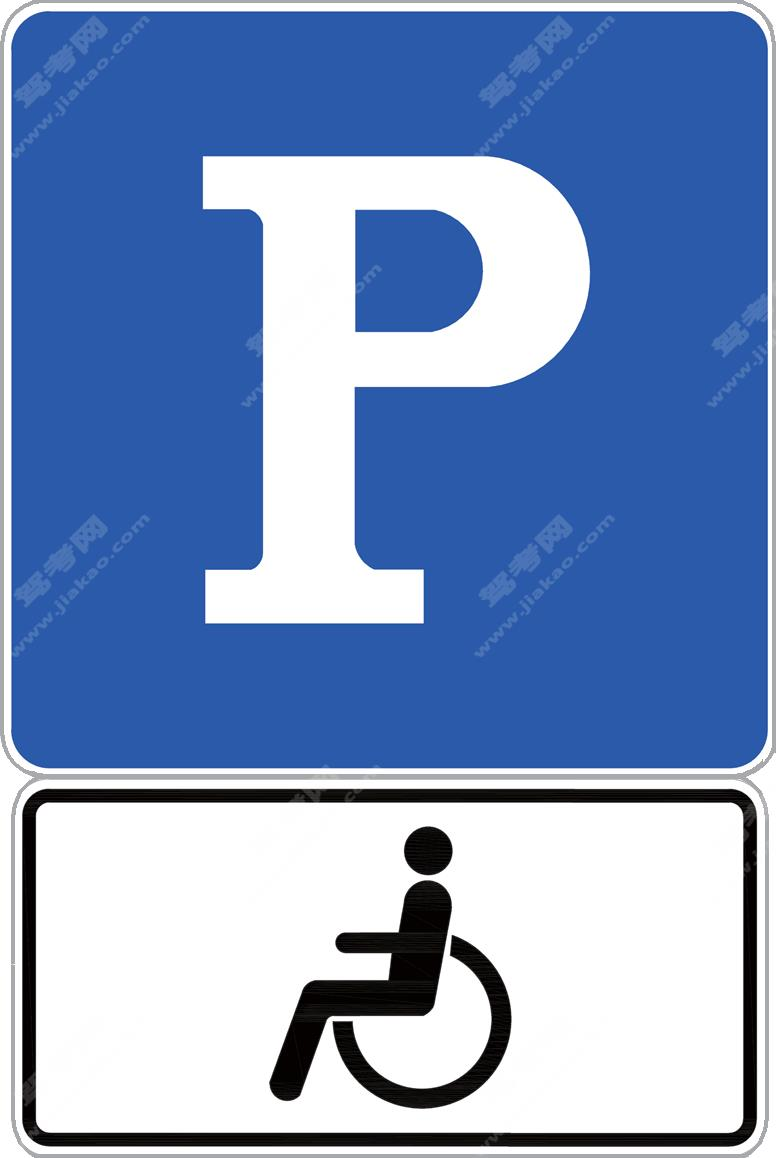残疾人专用停车位（新）

•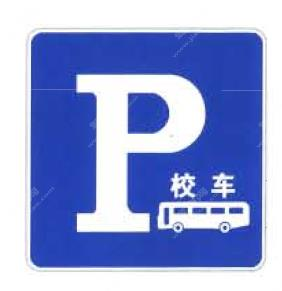校车专用停车位和停靠站点（旧）

•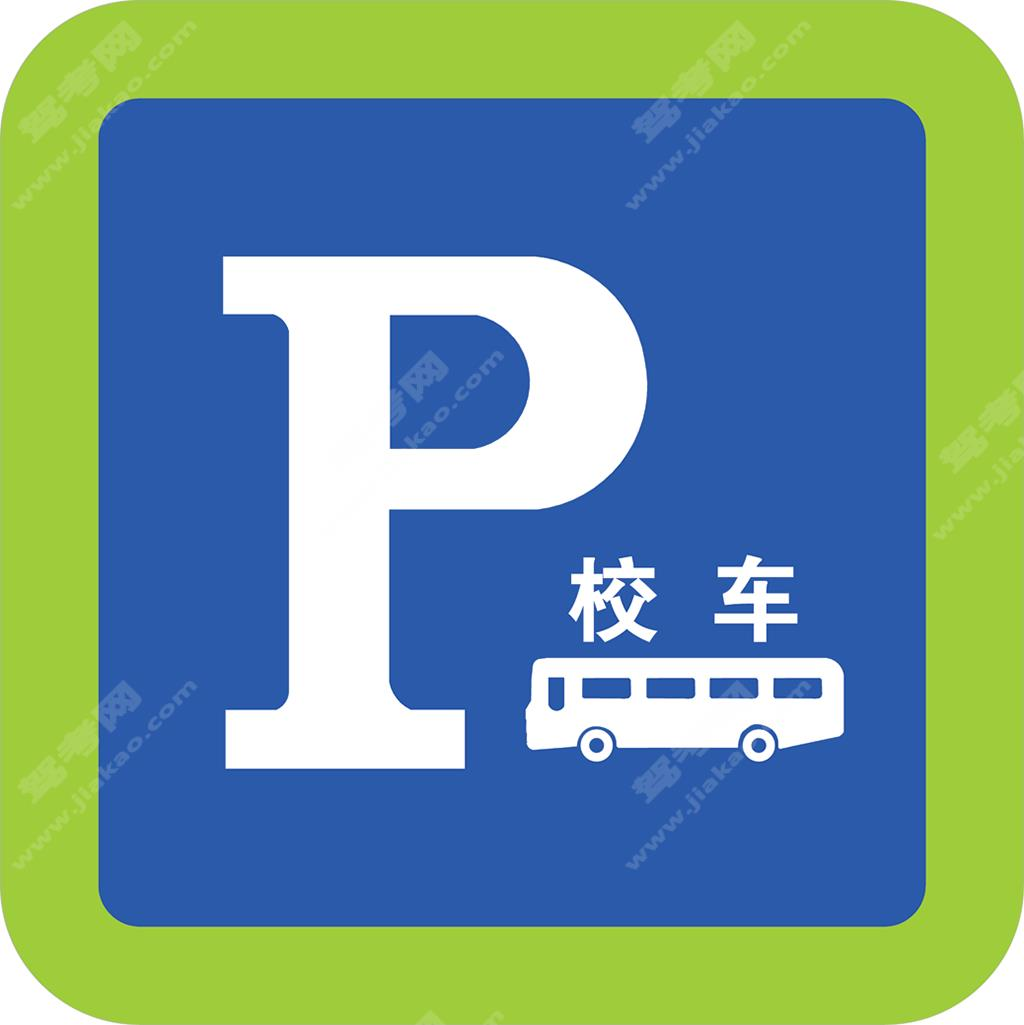校车专用停车位和停靠站点（新）

•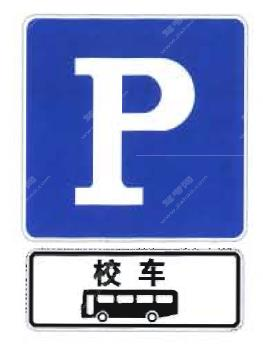校车专用停车位和停靠站点（新）

•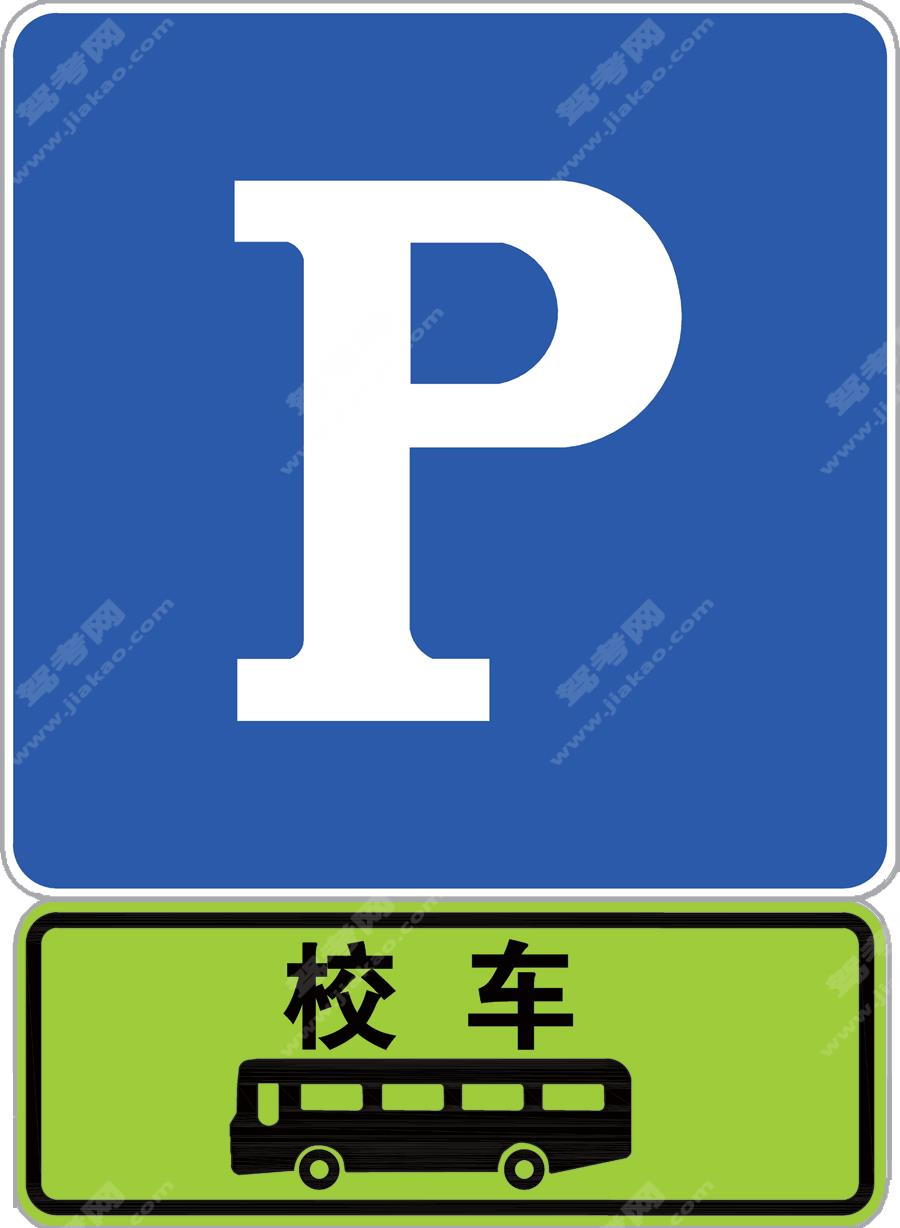校车专用停车位和停靠站点（新）

•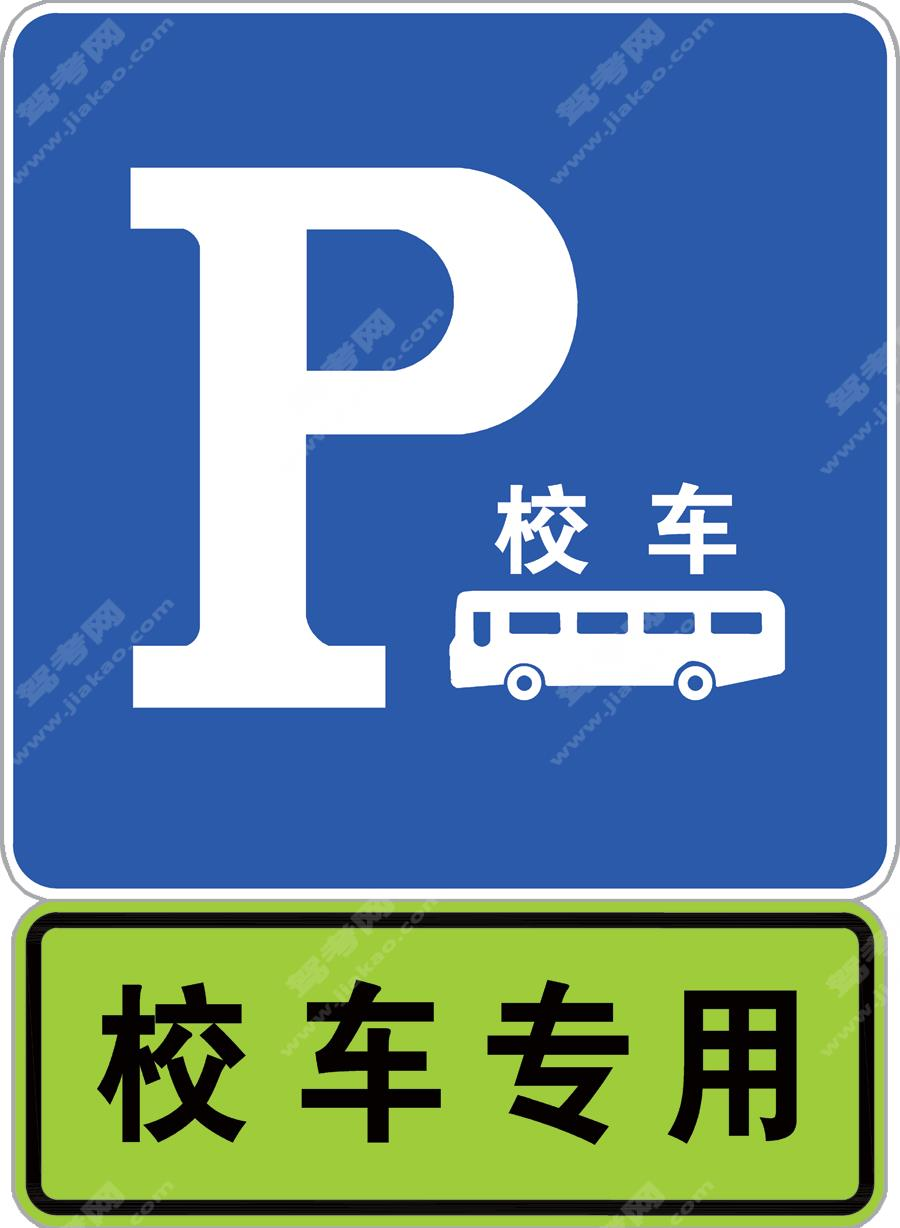校车专用停车位和停靠站点（新）

•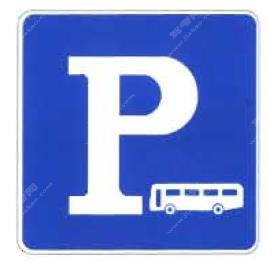公交车专用停车位（旧）

•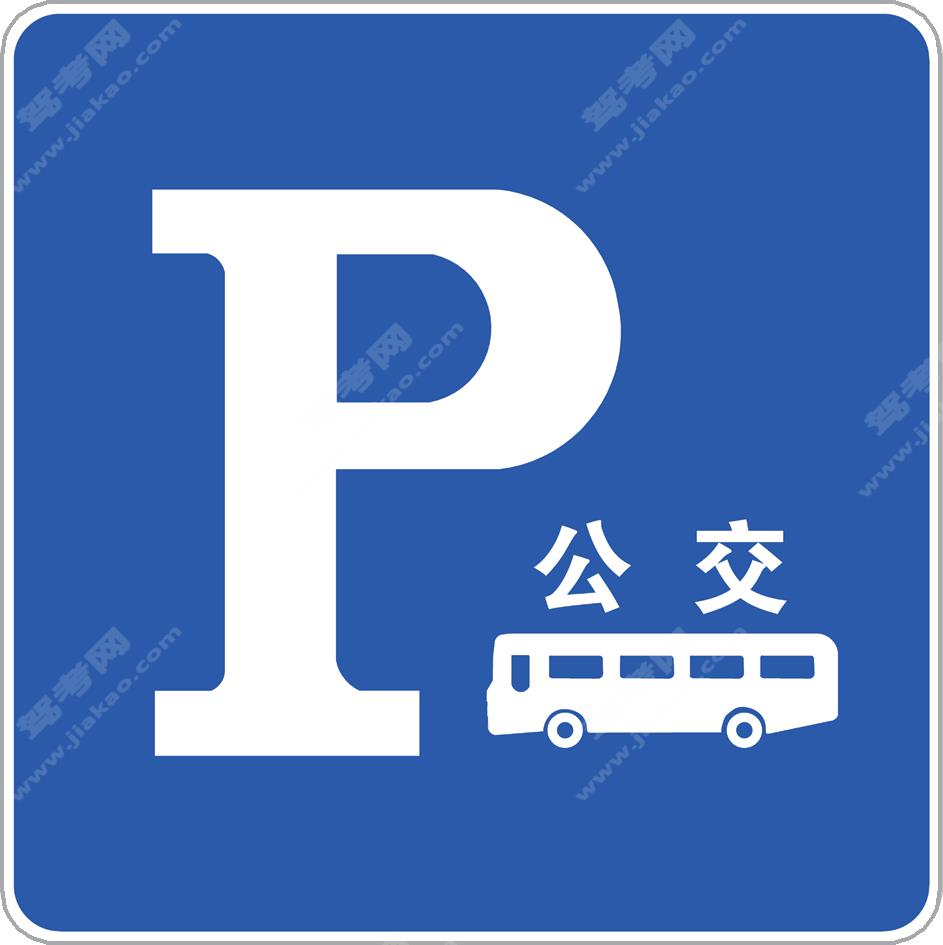公交车专用停车位（新）

•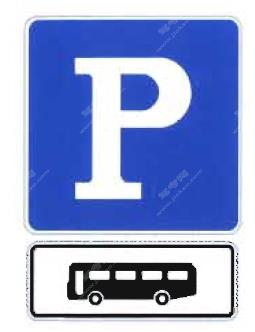公交车专用停车位（旧）

•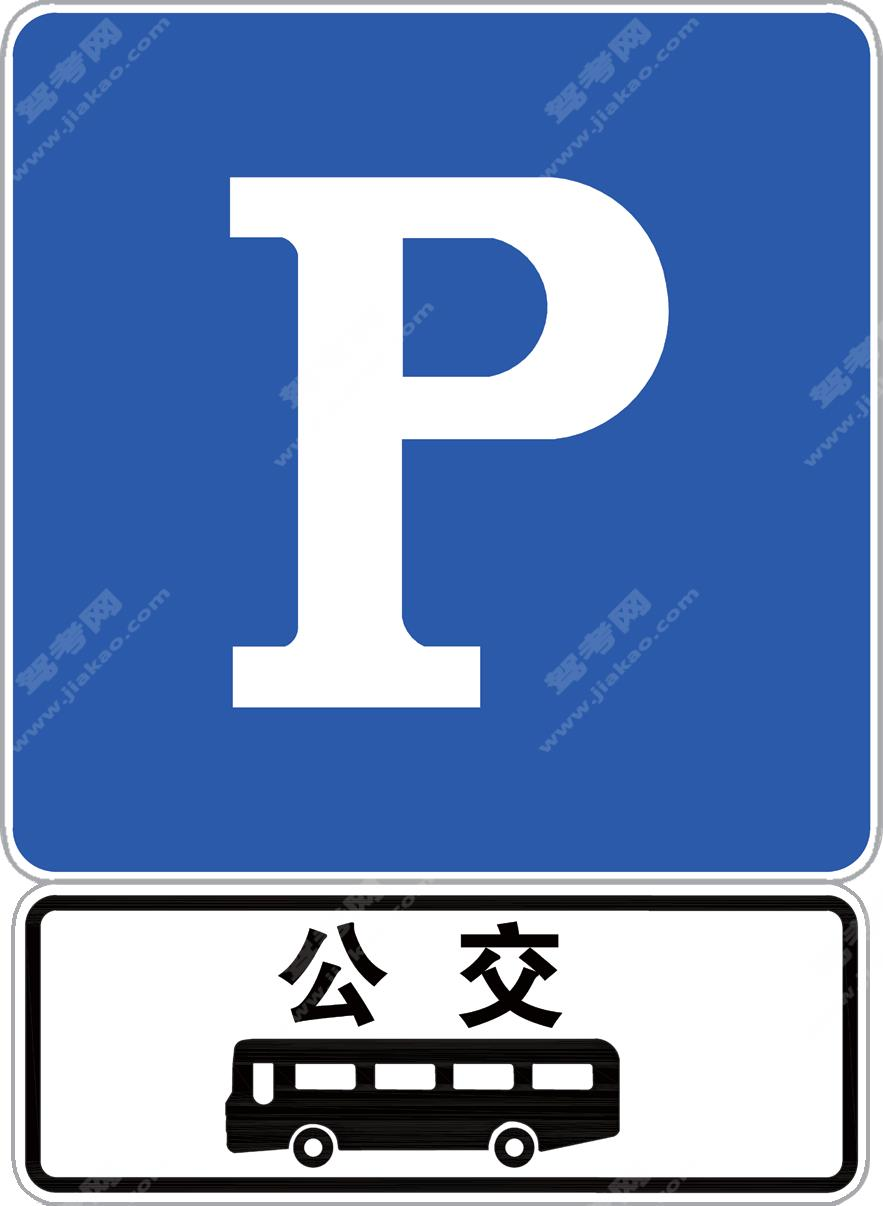公交车专用停车位（新）

•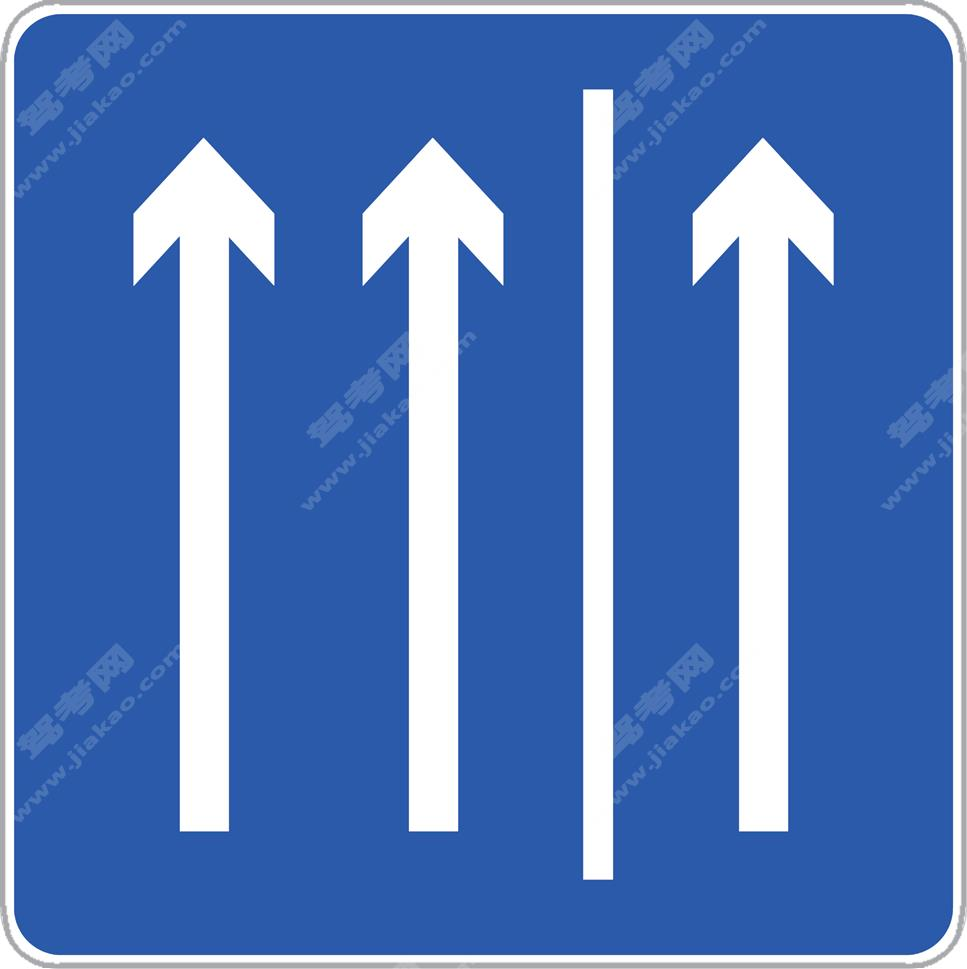硬路肩允许行驶起点（新）

•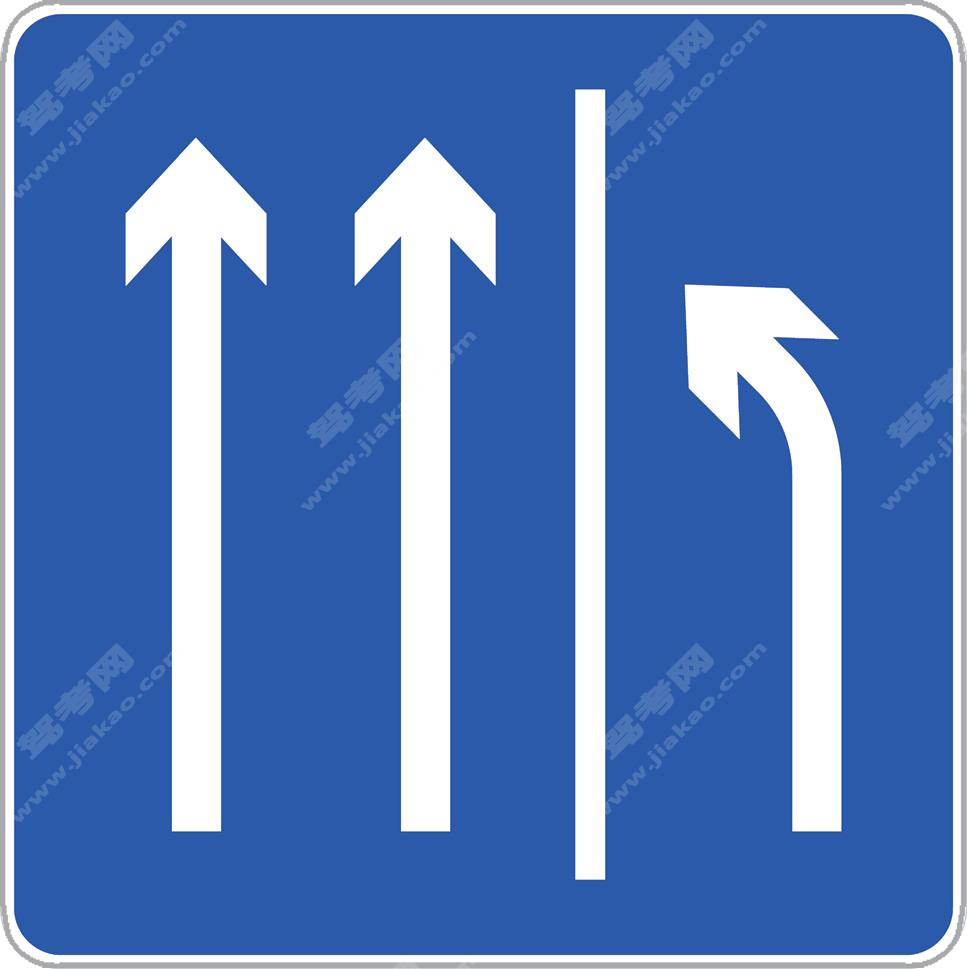硬路肩允许行驶即将结束（新）

•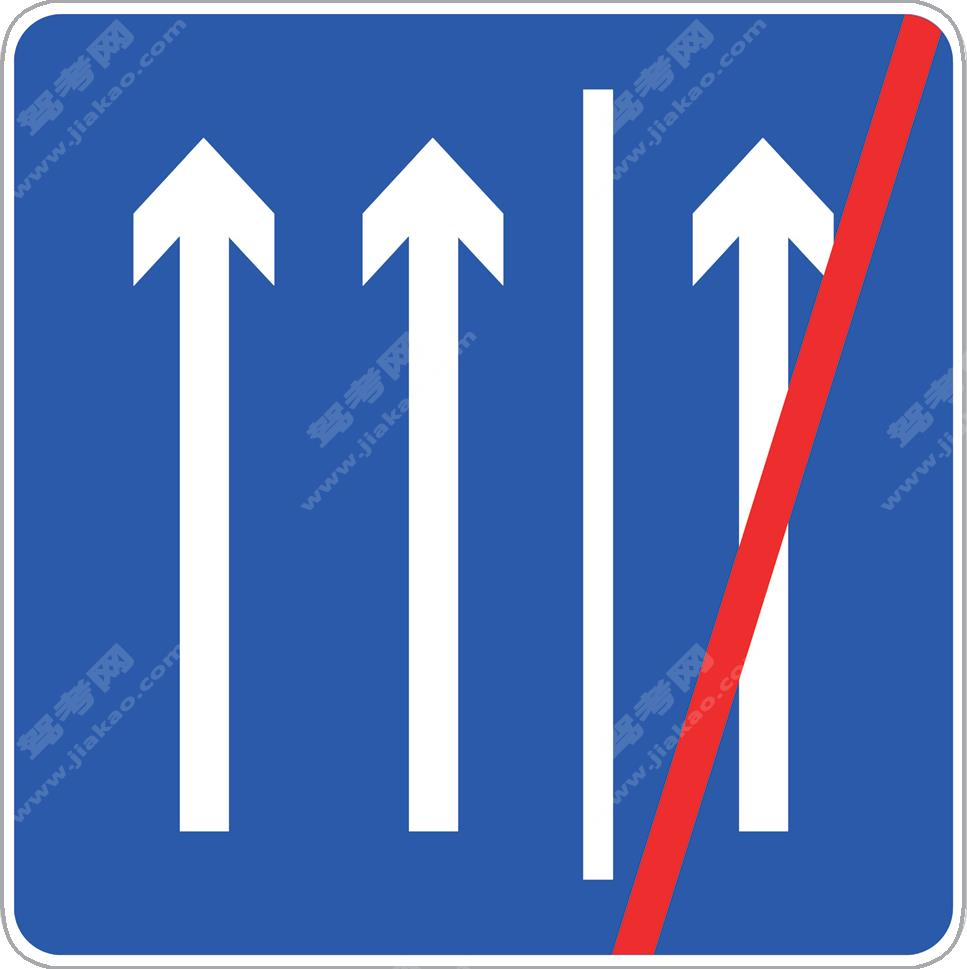硬路肩允许行驶终点（新）

•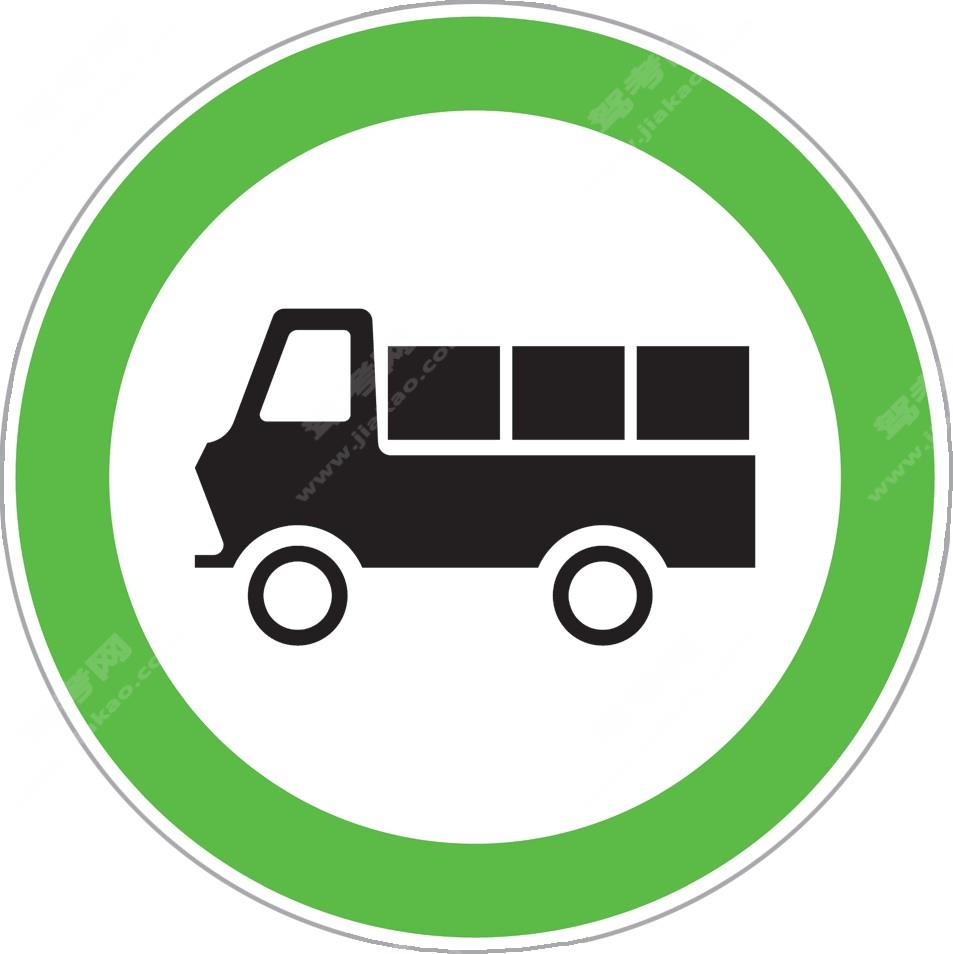货车通行（新）

•靠右侧道路行驶（删除）

•靠左侧道路行驶（删除）

•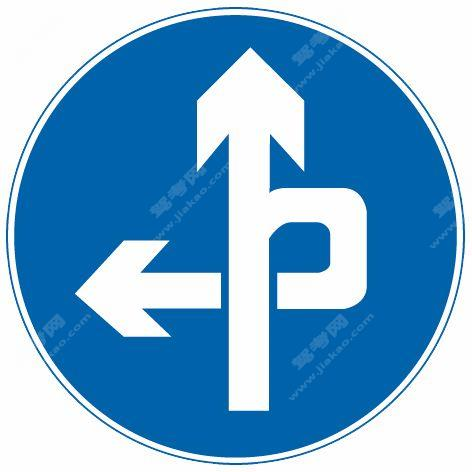立体交叉直行和左转弯行驶（删除）

•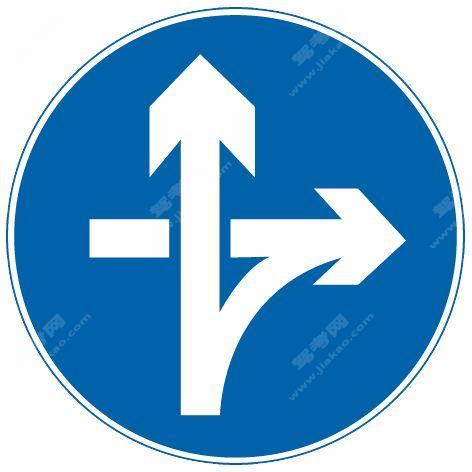立体交叉直行和右转弯行驶（删除）

•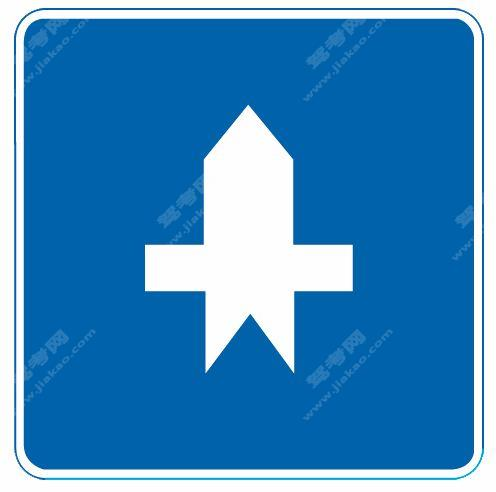路口优先通行（删除）

×